### Math 151 diary, fall 2006 In reverse order: the most recent material is first.

Wednesday, October 11 (Lecture #12)
Student volunteers (well, maybe they were impressed [one less often used meaning of "impressed" is "compel (a person) to serve in a military force") differentiated a bunch of functions.

I remarked:

1. I'll have a review session in our regular classroom on Sunday, October 15, at 5:00 PM. I asked that students take the opportunity seriously. Much of the material which is available has answers. Those answers should be read before attending the review session.
2. There is a review sheet of problems which will be gone over in workshop tomorrow.
3. We can only grade what students write on their exam papers. We can't grade intentions or thoughts or ... Please write carefully. As we grade the exam, we won't guess. We will read what you write.
4. Please do my problems. Read the exam questions with care and read the whole question. Don't "invent" and then try to answer your own question. Also please realize that I have some fragments of sanity, and therefore, since calculators are not allowed on the exam, if you find yourself doing a large amount of computation, the most likely Areasons are that you have invented your own problem (and thus will not be eligible for full credit, even if you solve it!) or you are not solving the problem correctly and efficiently.

## Some links to help students prepare for the exam

Here are some pages which have practice problems with answers completely worked out. You can glance at material you understand well, and test yourself. You can look at worked-out problems in other areas. This is the last full lecture on derivative formulas. Today we'll differentiate inverse functions. For technical reasons which will become clear as you study calculus and associated subjects, these functions are extremely important. They are important mostly because ... well, honestly, mostly because of their derivatives. This may seem weird to you, but please believe me.

arcsin
The text calls this function sin-1 and some other sources call it asin. I find the "-1" superscript awkward, and when I compute I may confuse it with "one over ...". So I'll call the function inverse to sine, arcsine, and abbreviate its value at x as arcsin(x). The "arc" part of the name refers to the angle whose sine is tangent, and, since we use radian measure here, angles are measured by the lengths of arcs they cut in the unit circle.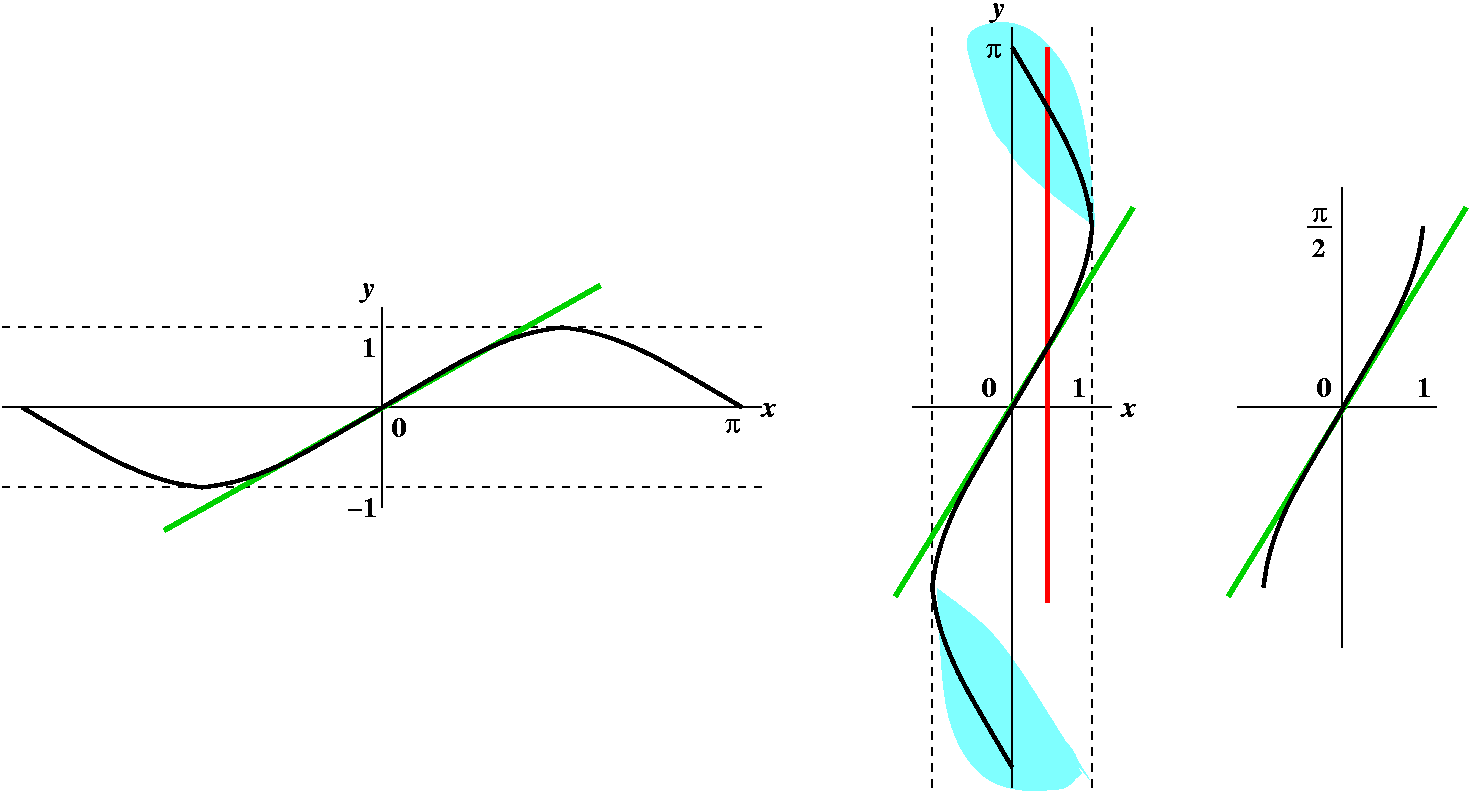What's happening in the pictures (left to right):
The first picture is supposed to be a portion of the graph of sine. It is 2Pi periodic, and its range is [-1,1]. The green line is the "main diagonal", y=x, which also happens to be tangent to y=sin(x) at (0,0). This is because the slope of the tangent line is the derivative of sine, which is cosine, and cos(0)=1. To get the inverse function, we interchange inputs and outputs. Geometrically we flip the graph over the main diagonal, and get the second picture. The tangent line is still tangent, but now, look at the red line. This demonstrates that the flipped graph is not the graph of a function. It fails the vertical line test to be a graph of a function. Thus we need to cut away (!) part of the graph. The "clouds" in blue-green (?) demonstrate what will be cut away. And what's left is shown in the third picture. This is the official graph of y=arcsin(x): domain [-1,1] and range [-Pi/2,Pi/2]. It has arcsin(0)=0, and the tangent lines seem always to slope up, so the derivative should be positive. And if we are very careful, the lines tangent to sine at +/-Pi/2 are horizontal, so the lines tangent to the flipped curve will be vertical and have no slope so there will be no derivative at +/-1. The derivative of arcsin should have domain (-1,1), the interval without endpoints.

Consider this process:

1. y=arcsin(x)   The inverse function
2. sin(y)=sin(arcsin(x))   Using the inverseness
3. sin(y)=x   Recognition of inverseness
4. cos(y)(dy/dx)=1   Implicit differentiation
5. dy/dx=1/cos(y)   Solving for dy/dx
6. Since sin(y)=x, x2+(cos(y))2=1, and so cos(y)=+/-sqrt(1-x2). Which sign to take? In the range we are considering between -Pi/2 and Pi/2, cosine is positive. Also the slope of the tangent line is positive for arcsine. Thus we will take the + sign. And cos(y)=sqrt(1-x2).   Solving for dy/dx stuff in x
7. Thus arcsin´(x)=1/sqrt(1-x2)   Statement of formula
We will go through this a few more times today. But in any case, the derivative is 1/sqrt(1-x2). Notice that for -1<x<1, this is positive, and that the derivative formula is not valid at +/-1 as the geometric evidence predicts.

Example: The derivative of arcsin(5x3-ex) is [1/sqrt(5x3-ex)](5·3x2-ex) using the chain rule.

arctan
The text calls this function tan-1 and some other sources call it atan. I will call the function inverse to tangent, arctan, and abbreviate its value at x as arctan(x).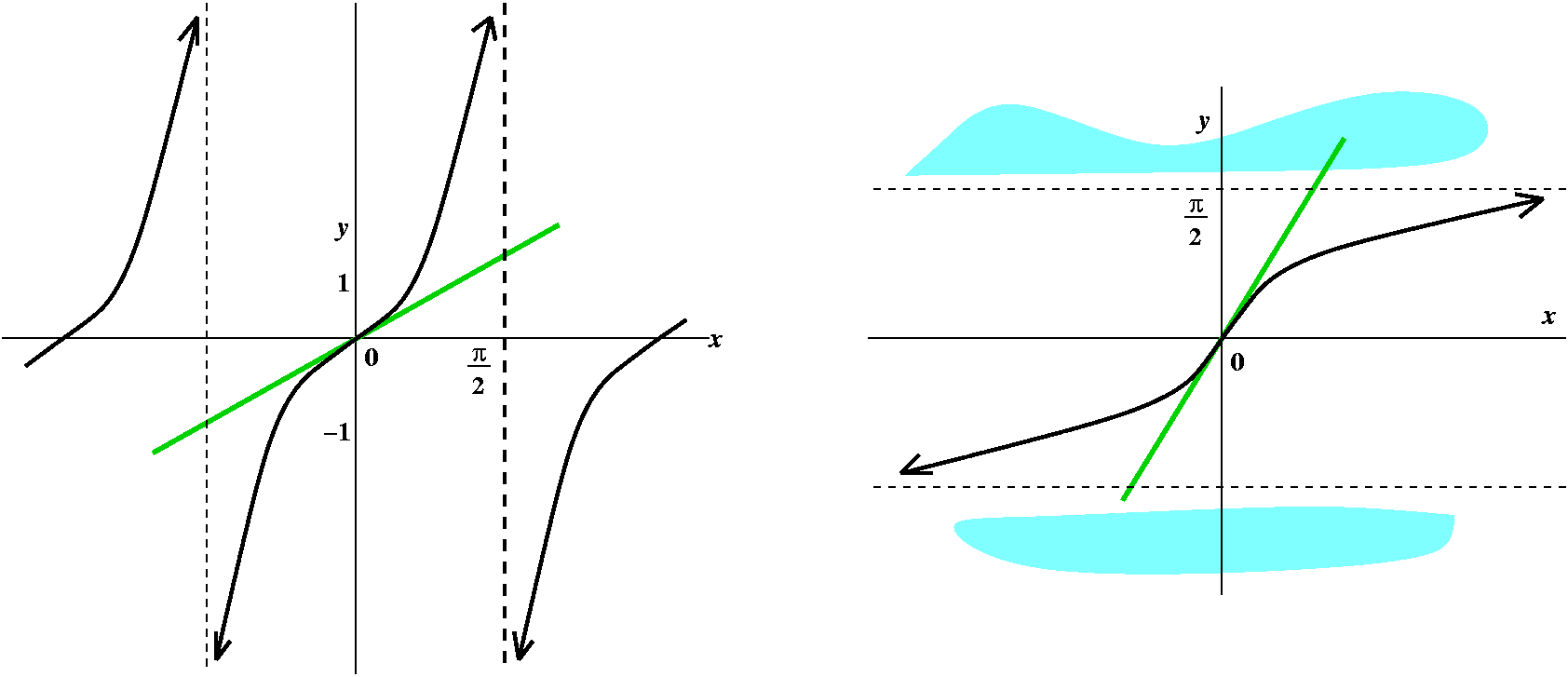What's the picture supposed to show? The initial picture is y=tan(x). This function is periodic with period Pi, and its domain does not include odd multiples of Pi/2. The function is rather simple looking (!), always tilted up, and has vertical asymptotes at odd multiples of Pi/2. Flipping to get an attempted inverse function reveals lots of problems (I omitted the red line here). The standard restriction is to throw out the "branches" that don't intersect the horizontal axis, and that's what I've attempted to suggest with the blue-green "clouds". Again, y=x is a tangent line to both arctan and tan at (0,0) because the derivative of tangent is (sec(x))2, and sec(0)=1/cos(0)=1. Arctan is very useful. It "compresses" all of the real numbers into the interval from -Pi/2 to Pi/2, so if you have lots of data and you don't know ahead of time how big (+ or -) the data will be, composing it with arctan will at least control it a bit. Now for the derivative.

1. y=arctan(x)   The inverse function
2. tan(y)=tan(arctan(x))   Using the inverseness
3. tan(y)=x   Recognition of inverseness
4. (sec(y))2(dy/dx)=1   Implicit differentiation
5. dy/dx=1/(sec(y))2   Solving for dy/dx
6. Since tan(y)=x, x2+1=(sec(y))2 (much easier than arcsine!).   Solving for dy/dx stuff in x
7. Thus arctan'(x)=1/(1+x2)   Statement of formula
Example: The derivative of arctan(e(5x4)) is 1/(1+(e(5x4))2· e(5x4)·5·4x3: somewhat of a mess, along with several uses of the chain rule.
The derivative of arctan([x+1]/[x-1]) is 1/(1+{[x+1]/[x-1]}2)·{[1(x-1)-(x+1)1]/[(x-1)22]}

The inverse function to exp (arcexp?)
Should this be called arcexp? Well, it isn't. The exponential function, ex, models exponential growth. It "takes" x and multiplies e's x number of times (can you understand that statement?). The inverse function to an exponential function is a logarithmic function. This logarithm function is very important. The log functions you may deal with include log10 (used in the definition of pH, and for hand calculation in "the old days") and log2 (used in some computer science applications). For reasons that will appear very soon, the log function which identifies how many powers of e appear in a number is called the natural log. Your text abbreviates it as ln, and that's what I will use, but note that many sources call it just log. What's ln? It is a function whose domain is all positive numbers and whose range is all real numbers.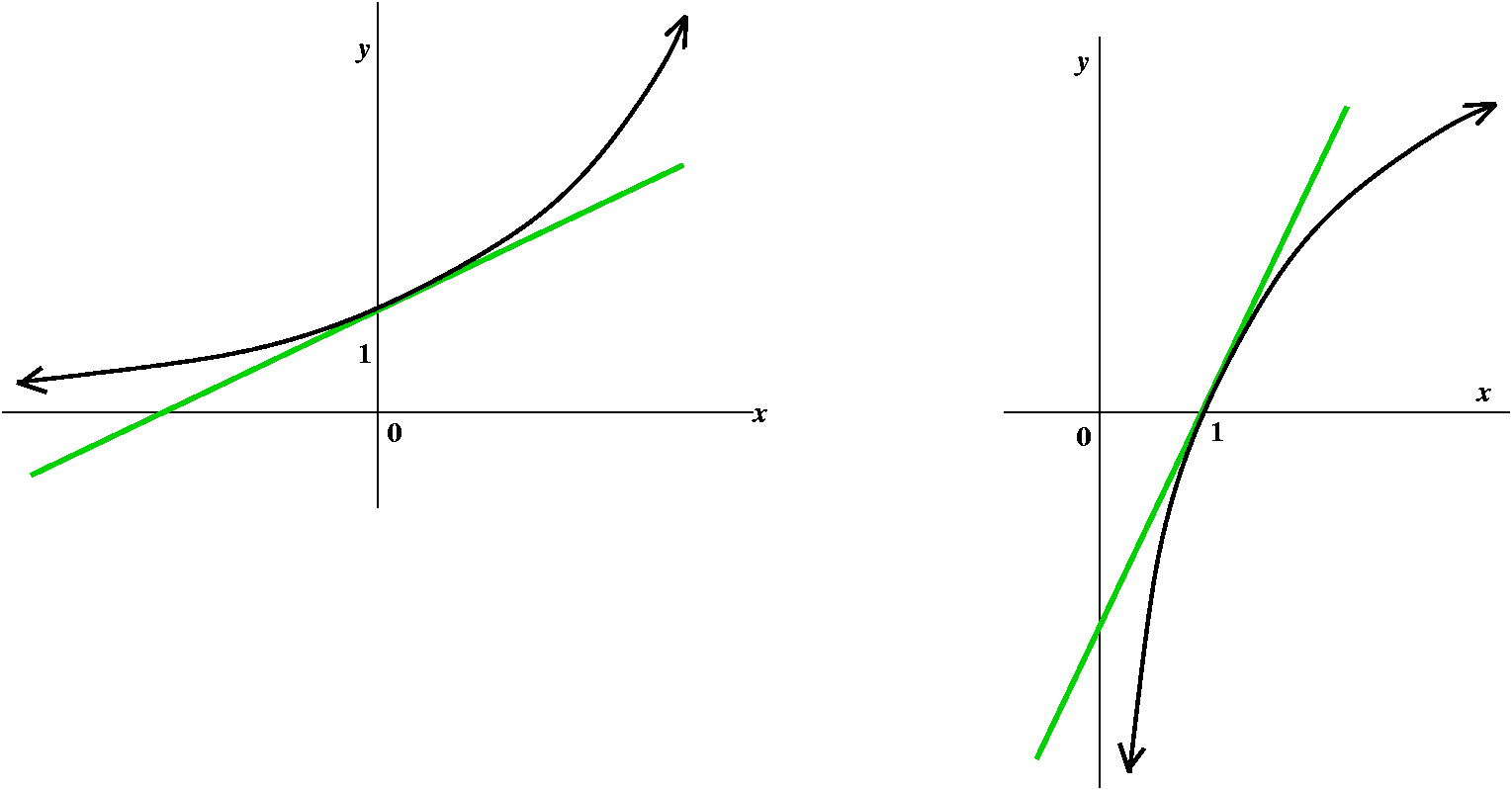This picture shows exp, the exponential function, ex. Since this function is one-to-one, its inverse will be a function: no more blue-green clouds! The green line is the tangent at (0,1) which has slope=1. Then we flip it and get the graph of ln. What about the derivative?

1. y=ln(x)   The inverse function
2. exp(y)=exp(ln(x))   Using the inverseness
3. exp(y)=x   Recognition of inverseness
4. exp(y)(dy/dx)=1   Implicit differentiation
5. dy/dx=1/exp(y)   Solving for dy/dx
6. Since exp(y)=x, we're done! This is even easier still   Solving for dy/dx stuff in x
7. Thus ln'(x)=1/x   Statement of formula

If y=ax is an exponential function, we could take log (really "ln") of both sides and get ln y= x ln a. Then we could differentiate both sides, remembering the chain rule. We would get (1/y)(dy/dx)=ln a (remember that a and therefore ln a are both constants!). Therefore dy/dx=y·ln a so that the derivative of ax is ax·ln a.
If we were interested in the derivative of 10x we would be looking at 10x(ln(10)). ln(10) is approximately 2.303 (this is enough for most purposes I know, but if you need more digits, here they are: 2.3025850929940456840). In computer science people sometimes consider 2x whose derivative is 2x(ln(2)). ln(2) is approximately .693 (again, enough information for most purposes I know, but ln(2) is .69314718055994530942 [more or less!]). The nicest and simplest ax for calculus purposes is one where the derivative is simplest, where ln a is 1. And that occurs when a=e. So

Of all the exponential functions,
ex is best
because it is its own derivative.

A differentiation technique you need for a calculus class
I differentiated y=xsin(x) by doing this:
Take log's (ln's) of both sides. The result is ln(y)=ln(xsin(x))=sin(x)ln(x) because ln(AB)=B ln(A).
d/dx the resulting equation carefully (implicit differentiation, product rule). The result of this is:
(1/y)(dy/dx)=(product rule!)cos(x)ln(x)+sin(x)/x
"Solve" for dy/dx, so that
dy/dx=y[cos(x)ln(x)+sin(x)/x]=xsin(x)[cos(x)ln(x)+sin(x)/x].

## even more Differentiation algorithms

FunctionDerivative
f(g(x))f´(g(x))·g´(x)
Implicit differentiation: given an equation relating x and y, d/dx the entire equation, and then solve for dy/dx. Be sure to use the chain rule and all of the other differentiation rules correctly!
arcsin(x)1/sqrt(1-x2)
arctan(x)1/(1+x2)
ln(x)1/x
axaxln(a)

Monday, October 9 (Lecture #10)

I then put the chart below on display and we discussed it for a while. Here's a pdf copy which you can print out separately if you like.

```
CHIPCO
INVESTMENT DOLLARS & PRODUCTION

Capital Invested    Chips produced      Marginal chips produced
\$ in millions       1,000's of units    1,000's of units per
millions of \$'s

200               3,000                    .23
300               3,040                    .28
400               3,070                    .42
500               3,100                    .78
600               3,190                    .31

CHIPCO
SALES & PROFITS

Chips marketed      Profit gained      Marginal profit
1,000's of units    \$'s in millions    Millions of \$'s per
1,000's of units

3,000                1.2                  .03
3,050                2.8                  .02
3,100                3.6                  .05
3,150                4.9                 -.01
3,200                5.1                  .02

```

I don't think many people were familiar with the economic terms used above. In particular, the word "marginal" is used in a fairly technical sense. In the first table above, it refers to the approximate amount would increase per each million dollars of increase in capital investment. Therefore, for example, if \$302 million were invested, then (according to this model) chip production would be 3,040,000 (chip production at the \$300 million level) plus .28(2)(1,000) chips. The 2 comes from the additional millions of dollars of capital. The 1,000 comes from the units I use for chip production. The .28 is this "marginal" quantity. In the first table, the marginal quantity is therefore the approximate amount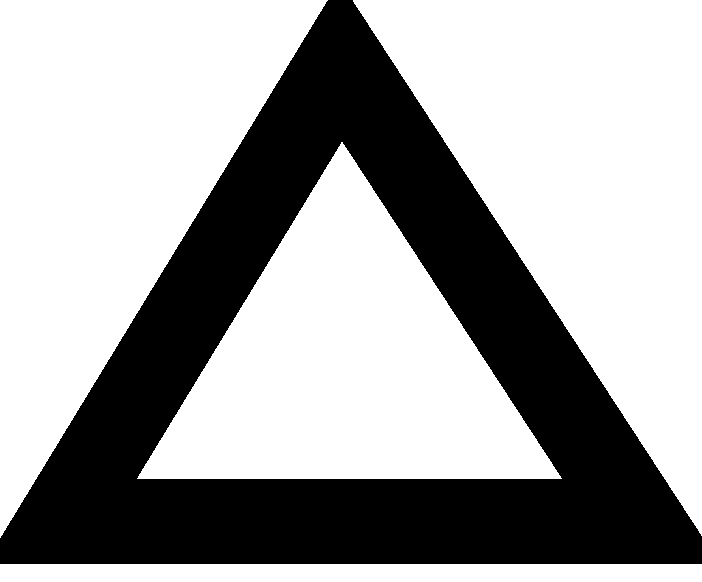P/C, relating the change in chip production to the change in capital investment. It is sort of a slope, or, more likely, sort of a derivative: indeed, the use of "marginal" in economics usually means a derivative. In this model, if \$297 million were invested, the approximate expected chip production would be 3,040,000 (again, chip production at the \$300 million level) plus .28(-3)(1,000). The novelty here is the use of the minus sign, since here we are decreasing the capital investment rather than increasing it.

The second table describes a similar phenomenon, here connecting the chip amount, C, with the profit derived from these marketing and sale of these chips. For example, the profit derived from the sale of 3,000,000 chips (the first line of the second table) is \$1.2 million. If we now look at the third column, the model predicts a marginal profit of .03 (in the given units). Using this, if 3,010,000 chips are marketed (that's 10 more 1,000 units of chips) the additional profit would be .03(10) million dollars, or \$300,000. And if only 2,970,000 chips were marketed, then the profit would be 1.2 million+(.03)(-30)(1,000)million. (I think I got all the units correct.) The third column givesP/C for various amounts of chip marketing: the change in profits compared to the change in chips marketed. Of course the validity of such models can certainly be criticized, but I really wanted to show this to explain what's in the next paragraph.

The two tables linked together describe a complicated phenomenon. First we "input" capital, M (M is for money), which produces C, a certain number of chips. Then the chips are marketed (and sold, hopefully!) to obtain a certain amount of profit, P. Here we have a composition of functions. For example, suppose we were asked how much profit there is if we put in M=\$500 million. From the first table we read off C=3,100,000 chips, and from the second table we can then see that P will be 3.6 million dollars.

I hoped that this was all fairly clear. Now I asked what I thought was a difficult question. Suppose we increase M from 500 million dollars to, say, 503 million dollars. What does the model predict the profit will be? We can trace this if we are sufficiently alert. The first marginal quantity we need to consider isC/M. For M=\$500 million, this is .78. So the new chip production is old chip production + increase in chip production, and this will be 3,100,000+(.78)(3)(1,000 chips). Now let us consider the chip/profit table. With C=3,100,000, we see that profit is supposed to be 3.6 million. But we are changing C by adding on the (relatively small) amount of .78(3)(in units of 1,000's of chips). The relevant marginal quantity here isP/C on the row where C is 3,100(,000). The marginal amount here is .05, so that the new profit will be the old profit (3.6 million) plus (.05)(.78)(3) million dollars. The 3 comes from perturbing the capital investment. The really interesting stuff is (.05)(.78): indeed, this represents the marginal profit as capital invested changes, when the capital investment is 500 million dollars. Symbolically, it might make sense written this way:P/M=P/C/M.
So theC's just seem to cancel out. Of course, this is more complicated than just multiplying fractions, since the fractions (the marginal stuff, the derivatives) need to be "evaluated" on the appropriate rows of the tables.

What I've done here is tried to present heuristic evidence that would allow us to believe the chain rule.

```
1. allowing or assisting to discover.
2. [Computing] proceeding to a solution by trial and error.
```
The Chain Rule Suppose that f and g are differentiable functions. The F(x)=fog(x)=f(g(x)) is differentiable, and F´(x)=f´(g(x))·g´(x).
Here o is supposed to be a little circle, and the little circle indicates composition. The tables above sort of indicated that chip production was a function of capital investment, and then that profit was a function of the chips marketed, so that profit as a function of capital investment was a composition of the two functions.

The balance of the lecture was devoted to exploiting the chain rule. There is a correct proof of the chain rule in the book. My first example was something like this (about as simple as I could imagine):
If F(x)=(x2+7)300, what will F´(x) be? I don't need the Chain Rule, not really (?), to compute F´(x) because, after all, F(x) is "just" a polynomial (although here F(x) is a polynomial of degree 600, and this polynomial is not presented in standard fashion). Success (rapid, accurate computation) here probably will result from recognizing that the chain rule applies.
If F(x)=f(g(x)), then g(x) is x2+7 so g´(x)=2x, and f(x) is x300 so f´(x)=300x299. Thus F´(x)=f´(g(x))g´(x)=f´(x2+7)(2x)=300(x2+7)299(2x). Whew!

But now comes the realistic comment. Hardly ever does anyone bother writing down all of these intermediate steps. That is, in practice very few f's and g's are actually identified. What happens is that people see and differentiate the outside most function (f above), put in the inner function (g) in that derivative, and then multiply by g´. For example, consider sin(ex+x2). What is its derivative? The outside function is sine, whose derivative is cosine. So I begin by writing cos(what's insidethe derivative of what's inside. The result is cos(ex+x2)·(ex+2x). This expression is a formula for the derivative of sin(ex+x2). Again, I urge you to consider the significance and necessity (!) of appropriate parentheses in these expressions. The "argument" of cosine is ex+x2 and the cosine expression is then multiplied by the expression (ex+2x).The chain rule itself can be repeated. So here, for example, we can try to differentiate cos(e3x2). Its derivative is -sin(e3x2)·(e3x2)·(6x). I hope that you can pick apart the layers of the functions and their compositions. One poor metaphor for using the chain rule is that it is like peeling an onion very very carefully, layer by layer, and taking care always of the outside most layer first. Confusion is certainly possible, and that's an understatement. The "chain" in the chain rule is, I think, a reference to the links (via composition) in a typical algebraically described function.

Here is an interesting application of the chain rule. Suppose we want to differentiate y=sqrt(x). Well (one student in the audience immediately and correctly said, (1/2)x-1/2) here is a way to do it. Square the equation to get y2=x and then differentiate the resulting equation. I will switch to what is called Leibniz notation now. Although this notation is not my favorite, somehow it fits with this sort of computation. In Leibniz notation, the derivative of y with respect to x is dy/dx, and what I want to do is d/dx (differentiate!) the equation y2=x. The right-hand side is easy: its derivative is 1. The left-hand side needs the chain rule: it has an "outside function", squaring, and an inside function, the "unknown function" y (unknown at least as far as its derivative is concerned). The result of the chain rule is 2y(dy/dx). Since this should be equal to 1, the derivative of the right-hand side, we can solve for dy/dx, and we get dy/dx=1/(2y). But y=sqrt(x), so we may recognize that the derivative of sqrt(x) is indeed (1/2)x-1/2.

This line of approach may be extended. For example, if y=x15/4 we then know that y4=x15. Then d/dx the result to get 4y3(dy/dx)=15x14 (do not forget the dy/dx which the chain rule forces upon you!). Then solve for dy/dx. It is (15x14)/(4y3). But since y=x15/4 we know that (15x14)/(4y3)=(15x14)/(4(x15/4)3)=(15x14)/(4x(15/4)·3)=(15/4)x14-(45/4)=(15/4)x11/4. Wow! A discovery: the power rule apparently holds for rational exponents. So the derivative of sin(x4/7) must be cos(x4/7)·(4/7)x-3/7.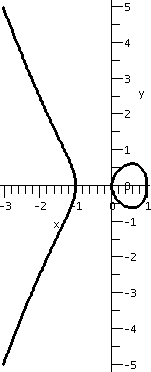The trick of having an equation involving x and y, then d/dx'ing the equation and solving for dy/dx is sometimes very useful. It is called Implicit Differentiation. There are times when it is difficult or impossible to get an explicit representation for y as a function of x. Then having an implicit expression is enough, if what is wanted is some information about dy/dx. I chose a rather strange example. Suppose we look at the equation x3+y2-x=0. The picture shown here is a result of the Maple command implicitplot which allows one to plot implicitly defined functions. Of course I was waiting for students to ask why the heck anyone would ever want to plot such a curve, and I picked the curve with some intent: this is an example of an elliptic curve, and cryptographic protocols related to such curves are extremely important today (Google has almost 3,000,000 references to elliptic curves!). If x=-2 in the equation x3+y2-x=0 we see that -8+2+y2=0, so that y must be +/-sqrt(6). What is the slope of the line tangent to this curve at the point (-2,sqrt(6))? We take the equation x3+y2-x=0 and d/dx both sides. The right-hand side gives me 0 (I always prefer to differentiate constants!) and the left-hand side is 3x2+2y(dy/dx)-1 which must therefore equal 0 (the chain rule forces the appearance of dy/dx). Inserting -2 for x and sqrt(6) for y, we get 12+2sqrt(6)(dy/dx)-1=0 or dy/dx=-11/(2sqrt(6)). Indeed, if you consider what the line tangent to the elliptic curve shown must look like, it does indeed slope "down".

Wednesday, October 4 (Lecture #9)
8
Students should be spending about eight hours each week outside of class really working on the material of the course.

L
The quiz was graded in a similar fashion to how the exam will be graded. Students should look at their results and be advised about the studying they need to do for the exam.

E
There will be an exam in 10 days. Both of the instructors will have review sessions the weekend before the exam. There will be sample material for students to see, also.

tenacity!
A former student (in several courses with me, about ten years ago) recommended that I quote this word to students. Well, tenacity means doggedness: persistent determination.

Reciprocals
If F(x)=1/g(x), then F(x+h)=1/g(x+h), and (F(x+h)-F(x))/h=[1/g(x+h)-1/g(x)]/h (this is a compound fraction, and I want to write it as a simple fraction, a quotient of two expressions) =[g(x)-g(x+h)]/[g(x)·g(x+h)·h]. Wow. With effort we can recognize the pieces. First, [g(x)-g(x+h)]/h-->-g´(x) as h-->0. And the "other stuff"--> 1/g(x)2 because g(x), since it is differentiable, must be continuous. This is a sort of reciprocal rule. Lots of work.

I remarked that if g(x) is getting bigger and bigger, I would expect the derivative to be positive. But then 1/g(x) would be getting smaller. The minus sign in the reciprocal rule algebraically shows this reversal.

I then computed the derivative of something like 1/x207. The result was -207x206/(x207)2. In fact some algebra can be productively performed on this, and we get -207x206-2(207)=-207x-208. Since the original function is 1/x207=x-207, we can see that the power rule also holds for negative integers. Recognizing patterns is an important part of mathematics, and a very important part of being usefully lazy.

I noted that the reciprocal rule allows the power rule to be extended to negative integer powers, so that the derivative of 1/x33=x-33 is (-33)x-34 or, equivalently, -33/x34.

Also we deduced the quotient rule: if F(x)=f(x)/g(x) where f and g are differentiable functions, then we can write F(x)=f(x)·(1/g(x)) so that (using the product rule and the reciprocal rule), F´(x)=f´(x)·1/(g(x)+f(x)·g´(x)/[g(x)]2. This is the quotient rule. The result, F´(x), is usually written as [f´(x)·g(x)-g´(x)·f(x)]/[g(x)]2. I did a some "simple" examples:

Moving right along (!) I discussed the trig functions again.Here are the standard definitions of trig functions as related to quotients of side lengths of right triangles:
sin(theta)=OPP/HYP and cos(theta)=ADJ/HYP and tan(theta)=OPP/ADJ. There are, of course, three other pairs of quotients, but need for them will be very rare in this course. Also included are two special triangles which give exact values of the trig functions at certain numbers (what is cos(35Pi/4)? what is tan(-103Pi/3)?)

Even more, we will need a kinetic view of the trig functions.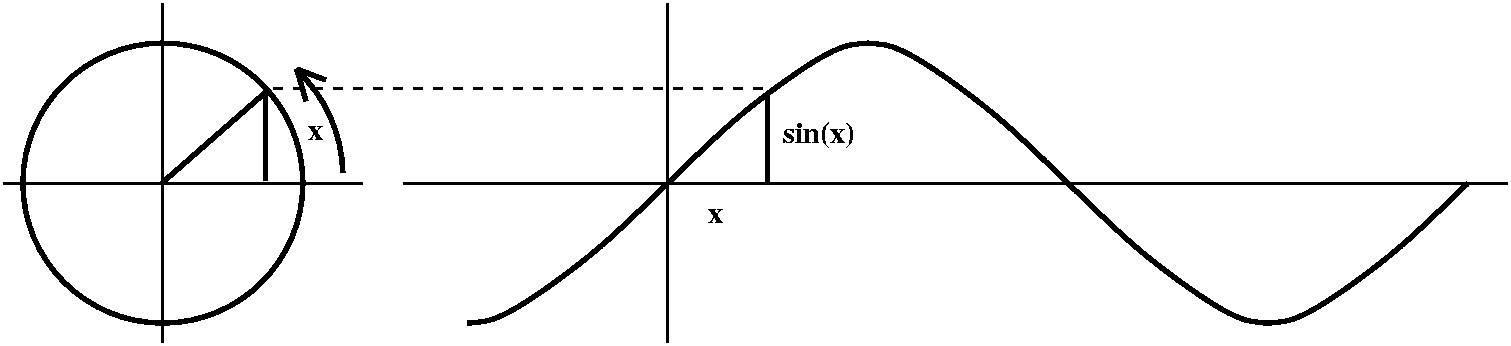If a point moves counterclockwise around the unit circle at unit speed, the second coordinate of the point is the sine of the time that the point has been traveling. The angle is measured by the length of the intercepted arc. In this scheme (radian measurement) the full circle of 360o is 2Pi radians. This is more natural if we want to consider periodic phenomena, like motion around a circle.

Sine: yet another sketch
I tried to draw an accurate picture of sine and then discussed what properties the derivative of sine would have.

• Where would the derivative of sine be 0? Well, where the tangent lines are horizontal. That should be at the tops and bottoms of the sine curve. These occur at Pi/2, 3Pi/2, -Pi/2, etc.: lots of places because sine repeats every 2Pi.
• Let's look carefully at sine between, say, x=-Pi/2 and x=Pi/2. I hope you "see" that the derivative, the slope of the tangent line, would start out at -Pi/2 at 0, then it would increase (as the tangent line began to tilt up). Then it would tilt up more (the slope would be more positive) until it would begin to tilt "down": here the language gets complicated. I am not asserting that the derivative is negative, but I am merely asserting that the slope, which stays positive, begins to decrease. Eventually the slope ends up, when x=Pi/2, at 0 again.
• What should happen between x=Pi/2 and x=3Pi/2, say? If your geometric "intuition" is superb, you can almost see that the curve I should draw on the derivative side starts down, so it is negative, gets more negative, then goes up, and ends up at 0. If you really see things very well, you may see that the shape of the derivative may exactly reflect the shape in the earlier interval, since the sine curve's shape is a flip of the earlier behavior.
• Etc. This word or abbreviation covers a lot. The derivative should be just as periodic as sine is. And it should geometrically be just as nice as sine.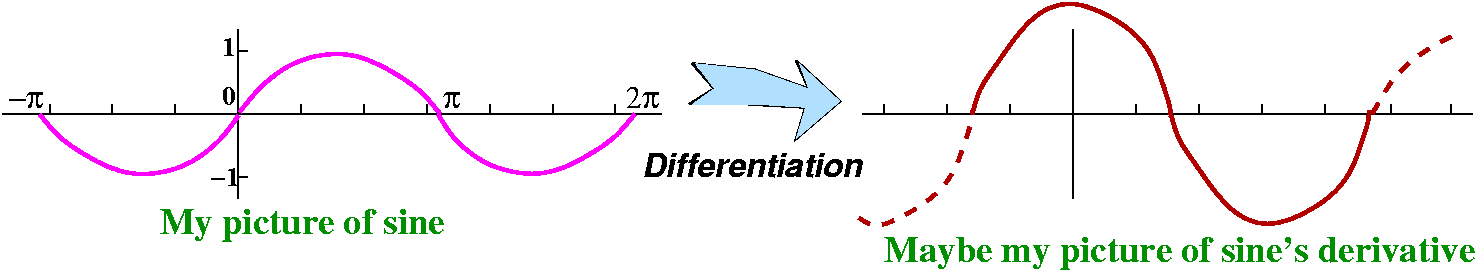Everything is arranged. Everything works out. What function has a graph which looks like the one drawn for the derivative of sine? Well, heck, we know such a function: cosine.

The derivative of sine is cosine.

In a calculus textbook, something like the following is done when f(x)=sin(x):

```
sin(x+h)-sin(x)     sin(x)cos(h)+sin(h)cos(x)-sin(x)
----------------- = ---------------------------------- = PIECE #1 + PIECE #2
h                          h```
where
```
sin(h)
PIECE #1 = cos(x) --------
h```
and as h-->0 this --> cos(x)·1 because we arranged it this way when we decided to use radian measure! Also,
```
cos(h)-1
PIECE #2 = sin(x) ----------
h```
If we multiply this top and bottom by cos(h)+1, the result on top is [cos(h)]2-1 which is [sin(h)]2. Then
```
[sin(h)]2             sin(h)               1
PIECE #2 = sin(x) ------------- = sin(x) -------- sin(h) ------------
h [cos(h)+1]             h             [cos(h)+1]```
Now as h-->0, I claim:
```
sin(h)                         1
sin(x)-->sin(x); ------ -->1;  sin(h)-->0; ----------- --> 1/2.
Nothing happens!      h                       [cos(h)+1]
```
So the result is sin(x)·1·0·1/2=0.
Don't worry. I believe this is the only time in the course I'll even write the addition formula for sine.Verification that limh-->0[sin(h)/h]=1
This is sometimes a handy thing to know. Also it is a limit which is often "requested" on calc 1 exams. Let me give you what I think is a fairly convincing discussion. I will look at the accompanying picture to the right. This picture shows a very small angle h, inside the unit circle (all of the radii are equal to 1).

• What is the area of triangle ABC? This is (1/2)base·height. The base is AB, which is a radius of the circle, so the length of AB is 1. The height is CD, the opposite side from the angle with measurement h and hypotenuse AC (another unit length, since another radius of the circle). Therefore sin(h) is the quotient of the length of CD divided by 1. So CD has length sin(h), and the area of triangle ABC is (1/2)sin(h)·1.
• What is the area of triangle ABE? This is (1/2)base·height. The base is AB, which is a radius of the circle, so the length of AB is 1.The height is EB, but EB/AB is tan(h), and the length of AB is 1, so EB has length tan(h). The area of triangle ABE is (1/2)tan(h)·1.
• What is the area of sector ABC? Here we need to think a bit. Several examples show what the formula for the area of a sector is:
The area of a whole circle of radius 7 is 72Pi.
The area of a half circle of radius 5 is 52Pi/2.
The area of a quarter circle of radius 3 is 32Pi/4.
The point of this was to convince the students that the area of a sector is directly proportional to the product of the square of the radius and the angle measurement (in radians). The constant of proportionality was (1/2): that is, the area of a circular sector of radius R and central angle Theta is (1/2)R2Theta.
Therefore the area of circular sector ABC is (1/2)12h=(1/2)h. (There's no Pi in this answer, because the Pi is already incorporated into the radian measure of the central angle!)
Now we see which of the areas is largest and which is smallest and which is middlest. (The sequence of letters "middlest" does not seem to be an English word. I am sorry.)

Smallest areaMiddlest areaLargest area
Triangle ABCSector ABDTriangle ABE
(1/2)sin(h)(1/2)h(1/2)tan(h)

Now the first two entries in the last row give us [sin(h)/h]<=1. The last two entries in the last row give us (after remembering that tan=sin/cos!) cos(h)<=[sin(h)/h]. Put them together:   cos(h)<=[sin(h)/h]<=1. We are interested in what happens as h-->0. Well, here is a valid use of version 1 of the squeeze theorem, since both cos(h) and 1 approach 1 as h-->0. So we can finally conclude that limh-->0[sin(h)/h]=1.

The function sin(h)/h occurs quite a bit when folks study vibrations of various sorts (vibrations in a beam or vibrations in an electric circuit or ...). Also the limit is sometimes really useful to know For example, on my "calculator", I just asked for sin(.0123) and got 0.0002146755. WHAT!!!??? Isn't this wrong? Isn't this way off? Well, no. I actually asked the calculator the wrong question. The calculator was set for degrees, not for radians. If you insist that your trig functions be all functions of degrees, then the derivatives will be all fouled up. In fact, the true value of sine of .0123 is actually 0.01229969, which is pretty darn close. So sine of h radians is darn close to h when h is small. And we can use this to compute other strange limits, if we have to.

Since limh-->0[sin(h)/h]=1 I know that limh-->0[sin(h)/(5h)]=5. And I know that limh-->0[sin(3h)/h]=limh-->0[3sin(3h)/(3h)]=3 since limh-->0[sin(3h)/3h]=1 (3h gets small along with h after all!)

I remarked that (looking again at the shape of the graphs) we can also see that the derivative of cos(x) is -sin(x) (there is a shift of Pi/2 in both graphs). Thus we get two more lines in the table of derivatives.

My final specific example of the quotient rule was to compute the derivative of f(x)=sin(x)/cos(x). Here top=sin(x) and bottom=cos(x), and the derivative will be (top'·bottom-bottom'·top)/(bottom)2. Notice that because of the minus sign, there is an asymmetry in the result, and this can lead to errors. f´(x)=[cos(x)·cos(x)-(-sin(x))·sin(x)]/[cos(x)]2. Here is an answer which demands simplification. The top is [cos(x)]2+[sin(x)]2 which is 1. The whole result is therefore 1/[cos(x)]2 or [sec(x)]2. Of course, this f(x) is tan(x), so we now know that the derivative of tan(x) is [sec(x)]2. Please note the parentheses. I almost always use lots of parentheses, because their use is almost required to make understandable the results of derivative algorithms.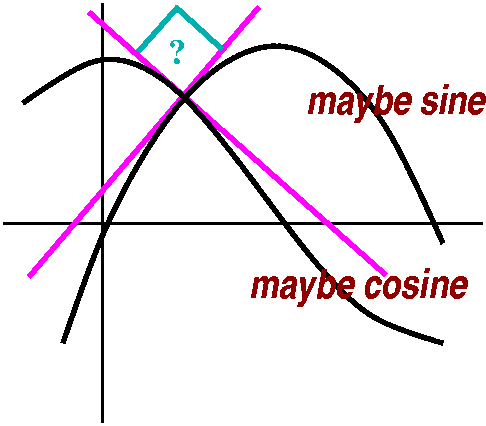My final gasp was to draw a quick picture, the way we all do, of sine and cosine. I distorted the bumps as almost everyone does. The bumps are actually rather flat. But "clearly" the curves intersect, and, look, look!, it seems that they intersect almost perpendicularly.
Can we check this? Well, sin(x)=cos(x) for x between 0 and Pi/2 when x=Pi/4 (that's the isosceles right triangle all the way up). Two lines will be perpendicular when the product of their slopes is -1 (or when "their slopes are negative reciprocals"). The slope of the line tangent to sine when x=Pi/4 is cos(Pi/4)=1/sqrt(2). The slope of the line tangent to cosine when x=Pi/4 is -sin(Pi/4)=-1/sqrt(2). The product of these two slopes is -1/2, not -1, so the curves do not intersect perpendicularly.

## more Differentiation algorithms

FunctionDerivative
1/g(x)-g´(x)/[g(x)]2
f(x)/g(x)[f´(x)·g(x)-g´(x)·f(x)]/[g(x)]2
sin(x)cos(x)
cos(x)-sin(x)
tan(x)1/[cos(x)]2=[sec(x)]2
EVEN MORE TO COME!!!

Monday, October 2 (Lecture #8)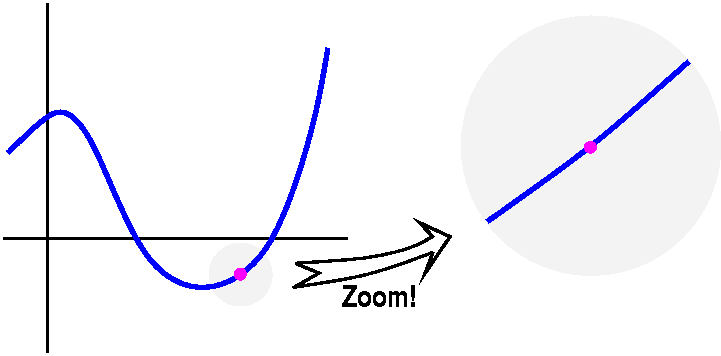• The official definition of derivative
limh-->0(f(x+h)-f(x))/h
• Derivative using a graph
A function will be differentiable if when you zoom in sufficiently on little piece of the graph around a point on the graph, the result looks very much like a straight line. A function whose graph looks like the graph of |x| at 0 is not differentiable, because no matter how much zooming is done, the result will not straighten out.
• The approximation property of derivative
The [REMARKABLE] equation I mentioned last time:
F(x+h)=F(x)+F´(x)h+Err·h
where as h-->0 then Err also-->0. Therefore the response to a perturbation h of the input is almost directly proportional to the input disturbance. The constant of proportionality is the derivative. If h is small, then you should think of the product Err·h is much smaller. Generally, experiments and measurements of various kinds observe the response f(x) and probably the additional term F´(x)h. The other stuff (at least when h is small) gets "lost in the noise" (hey, it is the noise!).
One useful result which can be deduced from the last statement is this:
In the equation F(x+h)=F(x)+F´(x)h+Err·h, as h-->0, then the second and third pieces of the right-hand side-->0, so that the left-hand side, F(x+h), must-->F(x). Therefore:

Every differentiable function is continuous.
Physically this says that if a point moves smoothly, it must move without any jumps or breaks.

The converse of this statement is not true. So there are continuous functions which are not differentiable (such as absolute value). Physically, there can be motion without any jumps but which is not smooth -- the particle can move with kinks and jerks.

The major topic for the next few lectures are the "classical" differentiation algorithms.

```
/algorithm/ n.
1. [Math.] a process or set of rules used for calculation or
problem-solving, esp. with a computer.

/alligator/ n
1. a large reptile of the crocodile family native to S. America and China,
with upper teeth that lie outside the lower teeth and a head broader and
shorter than that of the crocodile.

/allegory/ n.
1. a story, play, poem, picture, etc., in which the meaning or message is
represented symbolically.
```

It turns out that if a function is given by a formula involving the standard functions (powers, exponentials, trig functions, and their inverses) then usually the function will be differentiable, and the derivative can be written in terms of the standard functions. This is very nice. The methods, which I will show you, are mostly straightforward and have been implemented in numerous computer programs.

I started discussion of the differentiation algorithms. It turns out that for functions defined by generally simple formulas, there are a series of "rules" or algorithms which allow formulas for the derivatives to be written fairly easily. We will always start with the formal definition although it is comforting to recall such intuition as "f´(x0) is the slope of the line tangent to the graph of y=f(x) at x=x0" and that f' is also instantaneous velocity, etc.

I should have begun with the very simplest sorts of functions. If f(x)=15 for all x, then surely f(x+h)=15 also, so that f(x+h)-f(x)=0 and dividing this by h also results in 0, so the derivative is always 0. This result is true for any constant function. (Also the graph is a horizontal line, and is its "own" tangent line, with slope=0.) So we are done.

Now consider f(x)=xn. We need f(x+h) using the formal definition. f(x+h)=(x+h)n and we could use the Binomial Theorem to see exactly what the "expanded" version of f(x+h) looks like (see also information about Pascal's Triangle). But we actually don't need such precise information. For example, let's look at n=4. Here (x+h)4 is (x+h)·(x+h)·(x+h)·(x+h). We could multiply and expand everything or we could look at the structure of things a bit. There is exactly one product which is all x's, and that product has degree 4: x4. If we knock out exactly one x and take an h instead, we would have hx3. How many such terms can we get? Well there are 4 possible h's to choose, and we only want one of them, so there is exactly 4hx3 in the expansion. Every other term has at least two h's in it. We could "collect" all those and label them h2JUNK (junk because we won't have any need here of its precise nature). So:
(x+h)4=x4   +   4hx3     +     h2JUNK
All x's  Terms with 1 h    all other terms

We can do this more generally: (x+h)n=xn+nhxn-1h2JUNK
This is exactly a restatement, by the way, of the [REMARKABLE] equation I mentioned last time: f(x+h)=f(x)+f´(x)h+Err·h where you can "see" the higher-order error terms.

But now what? We consider limh-->0(f(x+h)-f(x))/h= limh-->0[(x+h)n-xn]/h= limh-->0[xn+nhxn-1+h2JUNK-xn]h= limh-->0h[nxn-1+hJUNK]/h= limh-->0nxn-1+hJUNK=nxn-1 which is in the table.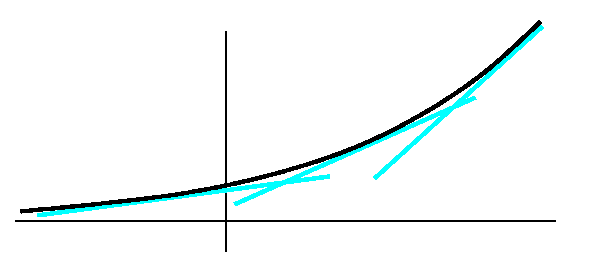Your textbook next studies the exponential functions ax. If a>1, this represents exponential growth. Consider the graph of an "average" exponential growth function and the slope of the tangent lines to this curve. As the point of tangency travels from left to right, the slope, which is always positive, just increases. If we could image a graph of the slope function (which is just a graph of the function y=f´(x)) it might look a great deal like ax itself. And that is the truth. A proof of this takes some effort, and could be given now, but we are supposed to run as fast as we can. So I will just write out some suggestive reasoning, following more or less what is in your text. I'll also include some pictures, mostly because pictures help me believe more.

If f(x)=ax, then f(x+h)=ax+h=axah. The difference quotient (f(x+h)-f(x))/h becomes (ah-1)/h multiplying ax. What is the number (ah-1)/h as h-->0? This number (the limit, if it exists, which it does) is sort of a "fudge factor" that's needed to make the derivatives come out right. -1<h<1
Investigation of the "fudge factor" occurring in derivatives of exponentials
a=2 Below are pictures of [2h-1]/h. The limit seems to exist, and its value seems to be about .693.
Thanks to M. Tsimaras for contributing a calculator graph in class. What's here is somewhat more accurate.
-1<h<1-.1<h<.1-.01<h<.01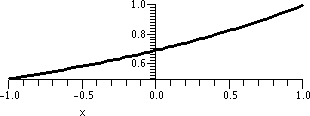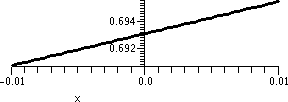a=2 Below are pictures of [3h-1]/h. The limit seems to exist, and its value seems to be about 1.09.
Thanks to E. Yi for contributing a calculator graph in class. What's here is somewhat more accurate.
-.1<h<.1-.01<h<.01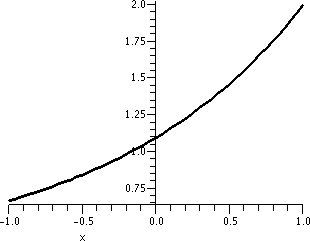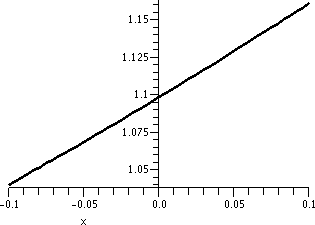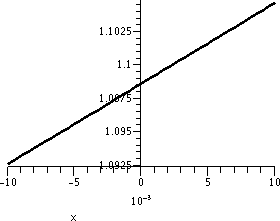n(1+{1/n})n
1 2.000000000
2 2.250000000
3 2.370370369
4 2.441406250
5 2.488320000
10 2.593742460
100 2.704813829
1,000 2.716923932
10,000 2.718145926
100,000 2.718268237
1,000,000 2.718280469
A similar exploration for the function 10x will result in a "fudge factor" of about 2.30. None of these numbers are too pleasant if you need to differentiate exponentials repeatedly (and most students will need to do this). So, let's see: 2x gives about .693 and 3x gives about 1.09. Maybe there is some nice number 2 and 3 for which the fudge factor is 1? Indeed, there is such a number. It is called e. It's approximate value is 2.71828...

e is defined to be the real number so that limh-->0 (eh-1)/h exists and is 1.
The immediate consequence is that
ex is its own derivative.
Of course this is a very nice, very convenient property. We choose e as the base for THE exponential function because it has the property that the local linearization near x=0 has slope 1. That is, we choose it so that the darn derivative will be as simple as possible.

I did not verify that there is such a number (e, that is). But the following "argument" provides one way to approximate it.

• Since (e1/100-1)/{1/100} should be approximately 1 we could multiply by 1/100 and see that (e1/100-1) is approximately 1/100.
• Now add 1 to both sides and see that e1/100 is approximately 1+{1/100}.
• If we took the 100th power (raised things to the 100) then the 1/100 and 100 in the exponent of e cancel (repeated exponentiations multiply) so that e is about (1+{1/100})100.

This is, by the way, 2.704813829. Well, you can see it in the accompanying table. This method of "computing" or approximating e is actually very very slow. The first several million digits of e are online, if you need them.

Then I worked on building new functions. If F(x)=f(x)+g(x), and the derivatives of f and g exist, what can one predict about the existence and value of the derivative of F?
Since F(x)=f(x)+g(x) we know that F(x+h)=f(x+h)+g(x+h), and the difference quotient for F can be written this way:
(F(x+h)-F(x))/h=[(f(x+h)+g(x+h))-(f(x)+g(x))]/h=[f(x+h)-f(x)+g(x+h)-g(x)]/h=[f(x+h)-f(x)]/h+[g(x+h)-g(x)]/h.
And now let h-->0, and we see that the derivative of the sum is the sum of the derivatives.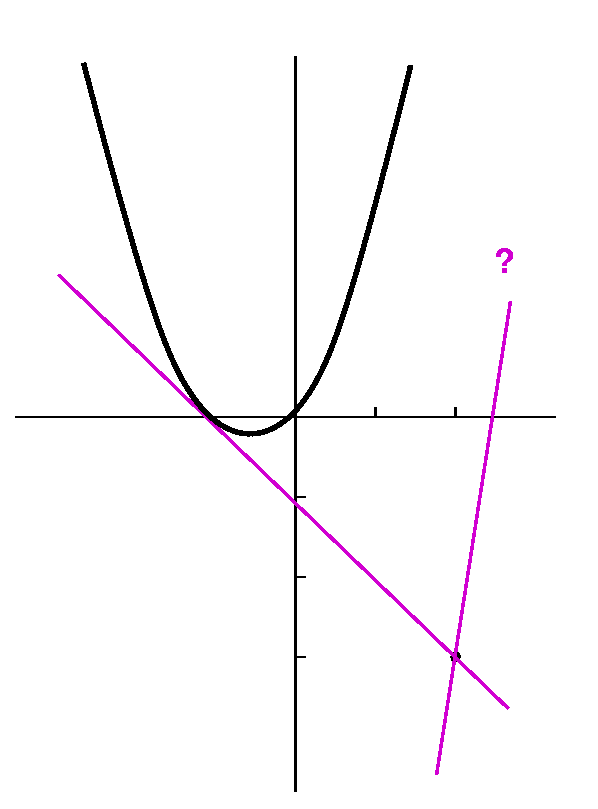Now I did a hard problem from the textbook (I think #50 of section 3.1), to show that we have gotten already to some level of achievement. The problem asks us to find the equations of the lines tangent to the parabola y=x2+x which also go through the point (2,-3). Note that although the problem statement does not request it, I would almost always begin the solution by making a sketch.

If P=(x,y) is the point of tangency on the parabola, we can solve the problem by realizing that the slope of the tangent line at P, mTAN, can be written in two different ways. First, since the tangent line goes through P and (2,-3), its slope is (y-(-3))/(x-2), which is (x2+x+3)/(x-2). But mTAN is also f´(x) if f(x)=x2+x. So mTAN=2x+1. Therefore 2x+1=(x2+x+3)/(x-2), and (2x+1)(x-2)=x2+x+3. Then 2x2-3x-2=x2+x+3 so that moving everything to one side, we get x2-4x-5=0. Since this is a problem in a textbook, the quadratic factors into (x-5)(x+1)=0. If x=5, then y=52+5=30 and the derivative is 2(5)+1=11. So the tangent line is y-30=11(x-5). We can make a cheap check: does this line go through (2,-3)? Well, -3-30=-33 and 11(2-5)=-33, so the answer is "Yes." The point of tangency is (5,30) which explains why we can't see it in the picture. You can find the equation of the other line yourself.

Now we began to discuss what is called the product rule. The statement of the product rule begins "The derivative of the product is ..." There is an expectation of simplicity and symmetry here, which should be eliminated as soon as possible. Consider x2 which is also, of course, x·x. The derivative of x is 1, and 1·1=1, but the derivative of x2 is 2x, so the product of the derivatives is not the formula we want.If F(x)=f(x)·g(x), then F(x+h)=f(x+h)·g(x+h), so that (F(x+h)-F(x))/h=(f(x+h)·g(x+h)-f(x)·g(x))/h. Now the game is to somehow write this fraction in terms of the difference quotient of f and the difference quotient of g. Here the picture may help. It tries to show a sort of decomposition of f(x+h)·g(x+h)-f(x)·g(x). The suggestion is that f(x+h)·g(x+h)-f(x)·g(x)=(f(x+h)-f(x))·g(x)+f(x)·(g(x+h)-g(x))+(f(x+h)-f(x))·(g(x+h)-g(x)). If we now divide by h and let h-->0, then:
[(f(x+h)-f(x))·g(x)]/h-->f´(x)·g(x) and [f(x)·(g(x+h)-g(x))]/h-->f(x)·g´(x).
The blue rectangle in the corner is a curiosity. It is algebraically (f(x+h)-f(x))·(g(x+h)-g(x)) (then divided by h) In the [REMARKABLE] equation I mentioned last time: F(x+h)=F(x)+F´(x)h+Err·h the blue rectangle belongs to the Error term. So what happens is this:
[(f(x+h)-f(x))·(g(x+h)-g(x))]/h=[(f(x+h)-f(x))/h]·(g(x+h)-g(x)). The first term -->f´(x) but the second term: g(x+h)-->g(x as h-->0 since g is continuous (because differentiable functions are continuous). Therefore the blue rectangle divided by h -->f´(x)·0 which is 0: it contributes nothing to the limit. This is a bit elaborate, but I'd like to be honest when I can be (!?). So now we know that the derivative of f(x)·g(x) is f´(x)·g(x)+f(x)·g(x). This is called the product rule or, sometimes, the Leibniz rule, memorializing one of the inventors of calculus.

Examples: If f(x)=x and g(x)=x, the product rule gives us 1·x+x·1=2x, the correct answer. We can also differentiate something like ex·x178. And we can differentiate 37·x23 with f(x)=37 (a constant function) and g(x)=x23. The product rule predicts that the derivative is 0·x37+37·23x22. Frequently people use this special case of the product rule without thinking about it: the derivative of a constant times a function is a constant times the derivative of the function.

## Differentiation algorithms

FunctionDerivative
f(x) limh-->0(f(x+h)-f(x))/h This is the formal definition
Constant0
xn when n is a positive integernxn-1
ex (Here e is approx. 2.71828)ex
f(x)+g(x)f´(x)+g´(x)
f(x)·g(x)f´(x)·g(x)+f(x)·g´(x)
(Const)f(x)(Const)f´(x)
MORE TO COME!!!

Wednesday, September 27 (Lecture #7)
Don't treat infinity like a number!
I had remarked that "statements" like
limx-->infinityf(x)=L
should be understood more as abbreviations of geometric descriptions than anything else. Therefore treating infinity as a number, adding or multiplying or whatever, may give "results" which are deceptive or even incorrect. I wanted to emphasize this and decided to show some examples using the following functions.
f(x)=x    g(x)=sqrt(x2+1)    h(x)=sqrt(x2+x)
I hope that you would agree with me that each of these functions have the property that, as x-->infinity, the function will also -->infinity. I mean specifically the following quantitative relationship:
If you choose any positive number M, no matter how large, then I can choose a positive number N so that if x>N, then the function will be >M.
For example, suppose I wish to exhibit this for the function g(x)=sqrt(x2+1). You tell me you want this function to be larger than 10,000. Well, if I want to force sqrt(x2+1)>10,000 then (square) this is the same as x2+1>100,000,000 and this is the same as x2>100,000,000-1. Well, let me "forget" the -1. If I only knew that x2>100,000,000 then x2>100,000,000-1 also. So how can I make x2>100,000,000? Just take x>10,000. So I can make g(x) big by making x big.

One example
I'm going to look at g(x)-f(x). This is sqrt(x2+1)-x. I want to investigate
limx-->infinity sqrt(x2+1)-x.
"Clearly" (always use that word when you can't explain where the idea comes from) multiply this by a fraction which has the same thing on the top and the bottom (so the fraction is a fancy way of writing "1".) Here the following fraction was suggested:

```sqrt(x2+1)+x
-------------
sqrt(x2+1)+x```
I was told that the top and bottom were each the conjugate of the formula for g(x)-f(x). Then
```                            (sqrt(x2+1)-x)(sqrt(x2+1)+x)
g(x)-f(x) = sqrt(x2+1)-x = ------------------------------- =
sqrt(x2+1)+x)

(x2+1)-x2               1
----------------- = ---------------
sqrt(x2+1)+x)     sqrt(x2+1)+x```
(Here we are using (A-B)(A+B)=A2-B2 with A=sqrt(x2+1) and B=x. The final fraction shows us that the result -->0 since the bottom grows to "infinity". Therefore limx-->infinityg(x)-f(x)=0.

Another example
Now let me look at h(x)-f(x). This is sqrt(x2+x)-x. I'd like to consider
limx-->infinity sqrt(x2+x)-x.
Now the appropriate conjugate is (sqrt(x2+x)+x).

```                            (sqrt(x2+x)-x)(sqrt(x2+x)+x)
h(x)-f(x) = sqrt(x2+1)-x = ------------------------------- =
sqrt(x2+x)+x)

(x2+x)-x2               x
--------------- = ---------------
sqrt(x2+x)+x)    sqrt(x2+x)+x```
Here things are bit more complicated.

Now look: sqrt(x2+x)=sqrt(x2[1+{1/x}])=sqrt(x2)sqrt(1+{1/x}). We've seen sqrt(x2) before, and what is it? If x is positive, then sqrt(x2)=x. (If x were negative, sqrt(x2 is -x.) Here we go:

```      x               x                              x                    1
-------------- = ------------------------- = ------------------- = -------------------
sqrt(x2+x)+x     sqrt(x2)sqrt(1+{1/x})+x     x[sqrt(1+{1/x})+1]    [sqrt(1+{1/x})+1]
```
Take a look at the last bit of "mess". The only appearance of x is in 1/x, and certainly as x-->infinity, this should-->0. All of the other pieces of the expression don't change. If you carefully examine them, you will see that the result is 1/2. Therefore limx-->infinityh(x)-f(x)=1/2.

Numerical evidence?
I haven't talked enough about numerical evidence for limits in class, mostly because I am scared of using a calculator or computer in front of people. But I certainly use such things on my own. So here is some numerical information.

x=10 x=102 x=103 x=104 x=105 0.0498756211 0.0049998750 0.0004999998 0.0000499999 0.0000049999 0.4880884817 0.4987562112 0.4998750624 0.4999875006 0.4999987500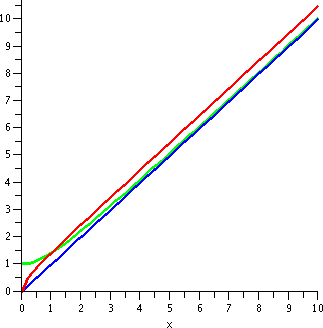I needed 25 digit accuracy to get the last few entries in the first row to 10 digit accuracy. Computations with small numbers which are almost equal can be imprecise. But the numbers should help you accept the previous results which were obtained with algebraic manipulation.

If you prefer graphical evidence then maybe the picture to the right can help. It shows graphs of f(x)=x and g(x)=sqrt(x2+1) h(x)=sqrt(x2+x) on the interval [0,10]. Although 10 is not very large, I think the asymptotic relationships between the functions are already apparent.

Short cuts are good but ...
So I know limx-->infinityx=infinity and limx-->infinitysqrt(x2+1)=infinity, but limx-->infinitysqrt(x2+1)-x=0.
And I also know limx-->infinityx=infinity and limx-->infinitysqrt(x2+x)=infinity, but limx-->infinitysqrt(x2+x)-x=1/2.
Please notice that 1/2 and 0 are not equal.
Therefore for this kind of limit, simple algebraic manipulations are not guaranteed to give valid results. We all like computational shortcuts, but limits involving infinity can be difficult to manipulate. Such limits should be treated more as descriptions of geometric situations rather that anything else.

Vocabulary?
On a well-known web page (concerning the Fungi of Australia, the word conjugate is defined to mean "copulation, especially isogamic copulation". I think I am too scared to look up "isogamic" since it might mean something illegal.

How to evaluate limits
As was remarked in the previous lecture, if the limiting function were defined with familiar formulas, I'd first try to "plug in" if there is any chance this strategy can be applied. If this simplest method can't be used, I'd try to "massage" the function (algebraically) and get to some algebraically equivalent restatement where plugging in can be used.

The derivative
Here is the most important single use of limit in Math 151.
Suppose f(x) is a function. Then we write f is differentiable at x=a if limh-->0[f(a+h)-f(a)]/h exists. If the limit exists, it is called the derivative of f at a and the notation f´(a) is used for the value of the limit.
Comments There are other notations for the derivative (almost every chunk of applied science and engineering has its favorite notation!). We'll see some of them. Please notice that the quotient involved in the definition of the derivative is exactly of the form which prevents direct "plugging in". If we insert h=0 in [f(a+h)-f(a)]/h we get [f(a+0)-f(a)]/0 and that's 0/0, a meaningless arithmetic expression.

One nice example
Suppose f(x)=1/x2. We already looked at this function in the lecture on September 13 (whose diary entry is not done, which I regret). Let's look at [f(a+h)-f(a)]/h:

```                        1         1
-------  - -----
1/(a+h)2-1/a2       (a+h)2      a2
----------------  = ------------------
h                 h```
My goal is to understand what happens to this as h-->0. If I "plug in" h=0 now I get no information. I will use part of my brain to manipulate this mess algebraically, and hope that I will eventually get to an equivalent algebraic form whose behavior as h-->0 will be apparent (by plugging in: that would be the easiest thing). Now what we have here is a compound fraction, and I will convert it to a simple fraction (with one "division"). Experience tells me that's easier to understand.
```    1         1          a2-(a+h)2
-------  - -----      -------------
(a+h)2      a2          (a+h)2a2          a2-(a+h)2
------------------- = --------------- = --------------
h                   h              h(a+h)2a2```
The major "transition" here is done by multiplying the top and bottom each by 1/h, and the resulting fraction has 1 in the bottom and is therefore a "simple" fraction. Now we expand part of the top, cancel a2 additively and h multiplicatively:
```  a2-(a+h)2       a2-[a2+2ah+h2]      -2ah-h2        h(-2a-h)      -2a-h
------------- = ---------------- =  ----------- = ------------ = --------
h(a+h)2a2        h(a+h)2a2          h(a+h)2a2     h(a+h)2a2    (a+h)2a2```
Now finally I can "plug in" h=0. More properly for this course, I can see what happens as h-->0. The limit does exist, and its value is -2a/{a2a2]. Usually people write this as -2/a3.
We have verified that f(x)=1/x2 is differentiable, and that f´(x), the derivative of f, is -2/x3.

Comments There are many opportunities to make algebraic errors in what's done above. One amazing thing is that we will develop very easy ways to compute derivatives for almost all familiar functions combined in interesting ways (including algebraic combinations and composition and "inversing"). Most of next week will be devoted to stating and understanding these results. Although you should (and will, darn it!) practice these rules (algorithms!), learning them is not the peak of the course. There are very nice programs which can compute such derivatives. A major purpose of the course is to understand why derivatives are interesting to people. We need to know how to use them. And maybe machines are not yet up to that level of cognition.

Not a nice example
Consider f(x)=|x|. I would like to see if this f is differentiable at a=0. Therefore I must consider:

```f(0+h)-f(0)    |0+h|-|0|    |h|
----------- = ---------- = -----
h              h         h```
I need to understand what happens to |h|/h as h-->0. Any computations with absolute value will go better if they are split into two parts, one from each side.

h<0 so we are considering h-->0- h>0 so we are considering h-->0+
Here h is negative, and |h|=-h. Therefore |h|/h is -h/h and this is -1: there's no appearance of h in this result. I think that the limit as h-->0- of |h|/h must be -1. Here h is positive, and |h|=h. Therefore |h|/h is h/h and this is +1: there's no appearance of h in this result. I think that the limit as h-->0+ of |h|/h must be +1.

But the limits from the two sides (+/-) don't agree. Therefore the limit limh-->0[f(0+h)-f(0)]/h does not exist.
We conclude that this function is not differentiable at x=0.

Interpretations
Here are a collection of simple interpretations of what we are discussing. Other interpretations will be shown in you in virtually every technical course you take from now on.

Math 151Geometry in the planeSimple physical motion
We're given some function f, and want to understand how it "changes". The object studied is the graph of f, which is the collection of points in the plane with coordinates (x,y) which satisfy y=f(x). Here we study rectilinear motion, where the position of a point on the coordinate line is given by f(x) (usually the variable is called t, not x, because this would help people remember that f(x) is the position at time "x").
The change in f over an interval from a to a+h is just f(a+h)-f(a). f(a+h)-f(a) denotes the difference in the heights of the function at the x-values a and a+h.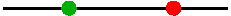f(a+h)-f(a) is the difference in position at the two times indicated. This is also called displacement. In general, this is not the distance that the point travels between those two times, because the moving point could wiggle back and forth.
[f(a+h)-f(a)]/h is called the average rate of change of f over the interval.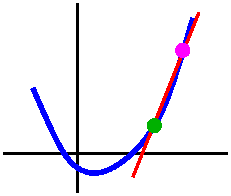[f(a+h)-f(a)]/h is the slope of the secant line through the points (a,f(a)) and (a+h,f(a+h)). [f(a+h)-f(a)]/h is called the average velocity of the point over the time interval.
If, as h-->0, the average rate of change of f approaches a limit, this limit is called the derivative of f at a and written f´(a).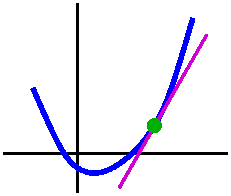If, as h-->0, the slope of the secant line approaches a limit, this limit is called the slope of the tangent line to y=f(x) at x=a. If, as h-->0, the average velocity of the point over the time interval approaches a limit, this limit is called the instantaneous velocity of the point at the time x=a. The limit in this case is frequently written ds/dt (difference in s, a Latin abbreviation for distance, divided by a difference in t, meaning time.

I will usually abbreviate the word "instantaneous" while discussing velocity, because I will almost never again refer to average velocity.

O.k.: a tangent line
Well, I made a mistake in class. I will not repeat the mistake here. Here I will use a different function. I will try hard not to make another mistake.

Suppose f(x) is the function defined by the formula sqrt(17+x3). Then it turns out that this function is differentiable (if you are "naive" this is not at all obvious and would be quite difficult to verify directly from the definition!) and f´(x)=[3x2]/[2sqrt(17+x3)]. Please: we will very soon see how this formula gets computed! Suppose I ask for an equation of the line tangent to the graph of this f(x) when x=2? We need some information.
A point on the line Well, (2,f(2)) is a point on the line, and f(2)=sqrt(17+23)=sqrt(17+8)=sqrt(25)=5.
Slope of the line Well, f´(2) is supposed to be the slope of the tangent line, and this is f´(2)=[3·22]/[2sqrt(17+23)]=3·4/[2·5]=6/5.
Therefore an equation of the line tangent to the graph of this f(x) when x=2 is (y-5)=[6/5](x-2). I am lazy and I will not "simplify".

Some numerical "work"
Very very very few of you will need to write the equations of tangent lines after getting through a first calculus course. But you will almost certainly need to consider many, many derivatives and make judgements based on these derivatives. What the heck is going on? Well, let us think about the example above. Since f´(2)=6/5, I know that
limh-->0[f(2+h)-f(2)]/h=6/5.
If I omit the "limh-->0" phrasing, then the equality is no longer ture. But the limit definition implies that what is true is a statement with an error term, and this error term is small when h is small. That is:
[f(2+h)-f(2)]/h=6/5+{ERROR [small when h is small]}. I can multiply by h and then add f(2) and get the following equation:
f(2+h)=f(2)+(6/5)h+{ERROR [small when h is small]}h

This equation is really why people study derivatives. The important qualitative aspect is that the Error is multiplied by h, and when h is small the Error is small. Products of "smalls" are even smaller, and the effect on the output of changing the input to f by h for small h is almost entirely determined by the multiplier, (6/5)h. Here are some numbers.

Input valuex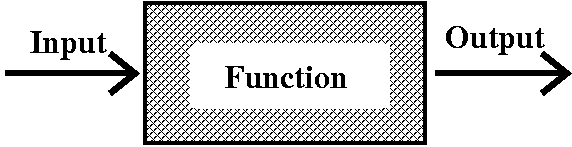x=2 f(x) is exactly 5. x=2+h f(2+h)=f(2)+(6/5)h+{ERROR [small when h is small]}h x=2.1 so h=.1 f(x) is about 5.124548 and f(2)+(6/5)(.1) equals 5.12 x=2.01 so h=.01 f(x) is about 5.012045 and f(2)+(6/5)(.01) equals 5.012 x=1.9 so h=-.1 f(x) is about 4.884567 and f(2)+(6/5)(-.1) equals 4.88 x=1.99 so h=-.01 f(x) is about 4.988045 and f(2)+(6/5)(.01) equals 4.988

Reinterpreting the definition
The function f is differentiable at a if for any very small {perturbation|kick|change} to the input, h, the output will be approximately f(a) (the old output) plus a constant multiplying the perturbation. That constant is the derivative of f at a.

### Monday, September 25

Diary entry in progress! Thursday ...
Students will need to hand in a writeup of a workshop problem and several textbook problems. They will also have a computational test about limits. An example of the test is available, as are answers .

The strategies available now for computing limits are:

1. "Plug in": if the function is given by a formula involving standard functions and you know the darn thing is continuous.
2. Transform what's given using standard algebraic manipulations into a form where you can "plug in".
3. There will be some other strategies, later.

Intermediate Value Theorem, restated
Suppose that the function f is defined and continuous on the interval [a,b]. Then the equation f(x)=y has at least one solution for every y which is between f(a) and f(b).

QotD, version 2 (fill in the blanks)
The QotD last time was not suitable. Here, maybe is something I should have asked, an alternative QotD for the last lecture, with a fill-in-the-blanks format.
Suppose f(x)=x3+cos(7x2+5)+sin(3x4-8)+2. Then f(-2) is  # 1  because (-2)3=-8 and the remainder of the formula describing the function f(x) is at most  # 2  at x=-2. And f(+2) is  # 3  because 23=8 and the most negative the remainder of the formula describing the function f(x) can be at x=2 is  # 4 . Since f(x) is  # 5  in the interval [-2,2] and the signs of f(x) at the endpoints differ, f(x) must have  # 6  root in [-2,2].

So the QotD would have been to fill in the blanks. Here we go: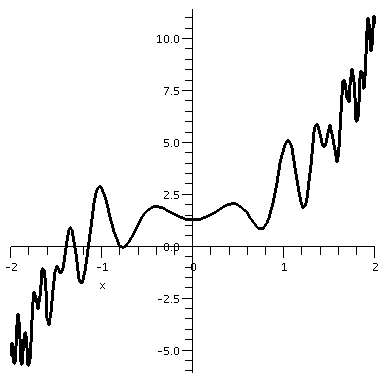1. negative
2. 4: the "outputs" of sine and cosine are between +1 and -1, and therefore cos(7x2+5)+sin(3x4-8)+2 must be somewhere in the interval [-4,4]. The "stuff" inside the sine and cosine terms is mostly irrelevant here.
3. positive
4. -4: the reasoning is the same as for #2.
5. continuous
6. at least one
To the right is a graph of y=f(x) on the interval [-2,2]. Indeed, there is "at least one" root inside the interval. Actually, there seem to be several roots.

Horizontal and vertical asymptotes: geometric and algebraic vocabulary
The limit idea was fairly successful, and people over years decided to use it to cover more and more situations. Sometimes the extensions were not as simple as the original setting. One extension that is useful describes certain geometric behavior of graphs called vertical and horizontal asymptotes.

Near x=3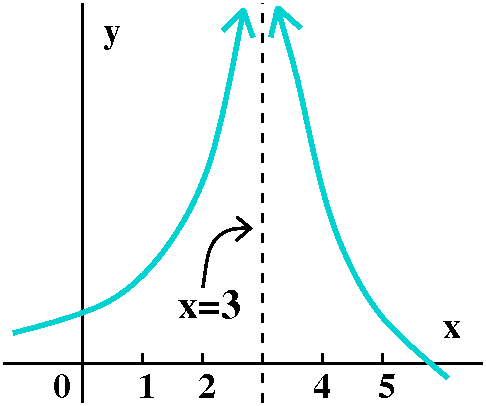To the right is the graph of a function, f(x), whose domain includes all x except for 3. The "arrows" at the top of the curve are supposed to indicate that as x gets closer and closer to 3 (on either the right or the left side) then f(x) gets large. But there's more, really. It doesn't just "get" large in some uncontrolled way. The function is supposed to

• Get as large as "you" want So if any number is named (10? 100? 100,000? 1010?), the function gets larger than that number close enough to 3.
• Stay large Not only does it get large, but eventually it stays large. For example, the graph is supposed to indicate that there is some (perhaps small) positive number, epsilon, so that if 0<|x-3|<epsilon, then f(x)>1010. This is, for me, difficult to imagine.
If both the "get large" and "stay large" statements are valid, then x=3 is a vertical asymptote of f(x). And, further, this geometric and numerical situation is abbreviated algebraically by
limx-->3f(x)=infinity.
I add immediately that I am not declaring that "infinity" is a number. I am merely telling you that this particular limit statement is supposed to be an abbreviation of the geometric situation shown.

Examples
1/(x-3)? 1/|x-2|?? 1/(x-3)2??? 1/(x-3)EVEN POS INT???

A rational function
x2/[(x+2)3(x+4)6]

Horizontal asymptotic behavior
Be a bit careful ...sin(x)/x
Not damned oscillation, just damped oscillation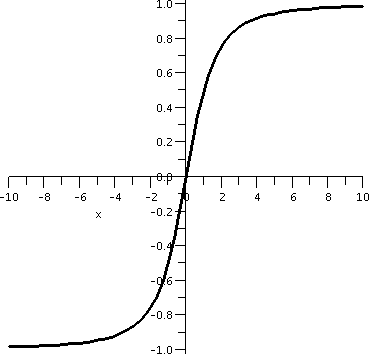x/sqrt(x2+3)

### Wednesday, September 20

Diary entry in progress!

Review of the definition of limit

Framework of ideas supporting the definition of limit

Algebra

Order (1)

Wiggling

Squeezing the wiggling

Order (2)

Plugging in

An important official word: continuity

The Garden State Parkway, from Cape May to Montvale and my friend Francine ...The Garden State Parkway runs most of the length of New Jersey. Mile 0 is at Cape May, while the other end, mile 172, seems to be close to Montvale. Suppose that my friend Francine leaves Cape May at 7 AM one morning, and drives north on the Garden State Parkway. Further, suppose she arrives at mile 172, the northern end, at, say, 10 AM. Must Francine at some time be at mile 135 (fairly near Busch campus)? The parkway seal here was "borrowed" from a State of New Jersey webpage.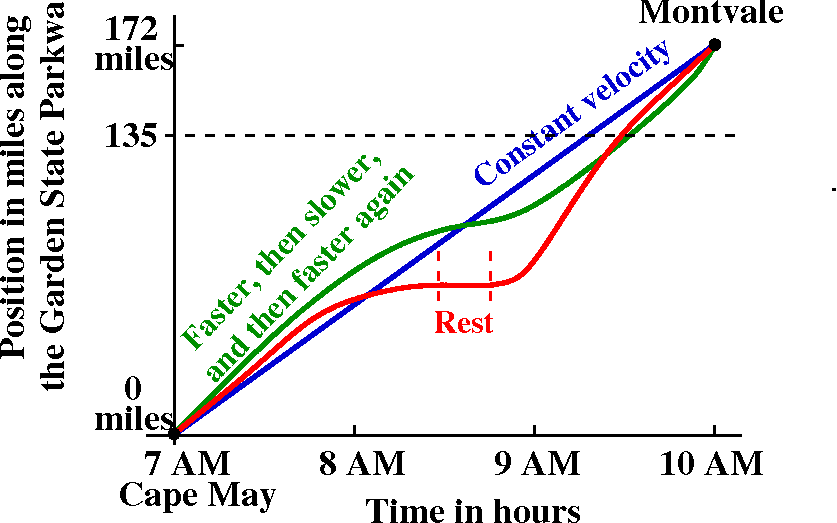We discussed various curves which could represent the position of Francine on the parkway in terms of miles from the start of the parkway at time t, in terms of hours elapsed from 7 AM. I tried to show that our everyday intuition lead to the graph being increasing (as you travel from left to right, the points on the graph go up). The graph can have level spots, where Francine pulls over for a rest stop. Legally Francine isn't supposed to drive backwards, though.

If we believe that motion is continuous (so Francine does not have a Star Trek transporter or other device) then the graph of Francine's position goes from (7 AM, 0 miles) to (10 AM, 172 miles) and therefore the graph must have on it at least one point with coordinate description (*,135). All of this, by the way, rests on some complicated assumptions, some of them philosophical (why should motion be continuous?). Today, though, I believe that motion is continuous, and therefore at sometime Francine must be at Mile 135. By the way, I will retain this information for later, when we analyze the rate of change of position (velocity) so that we can see whether Francine deserves a speeding ticket.

The Intermediate Value Theorem
Suppose that the function f is defined and continuous on the interval [a,b]. Then the equation f(x)=y has at least one solution for every y which is between f(a) and f(b).

In mathematics, the word theorem is applied to results that are deduced from basic principles, and usually the term is used for more important conclusions in the subject. In this case, the Intermediate Value Theorem follows from basic principles governing the real numbers. A particular basic principle which is used in the proof of the theorem is the "least upper bound" property of the real numbers. This essentially declares that there are "no holes" in the real numbers. A precise statement is fairly delicate, and this property essentially shows that the reals and the rationals are distinct. Several upper-level math courses spend quite a bit of time exploring the statement. You can read about it in Wikipedia but I do add that detailed knowledge of such foundational material is not needed for success in Math 151 (or, for that matter, for successful careers in almost all of science and engineering!).

The square root of 2
If we were desperate to compute sqrt(2) (that is, really, desperate to approximate sqrt(2)), for example, we could look at f(x)=x2-2 on the interval [0,2]. This f(x) is certainly continuous. (We already observed that we could "plug in" values to evaluate limits for polynomials. I know that f(0)=-2<0 and f(2)=+2>0. Therefore according to the Intermediate Value Theorem there will be at least one x inside the interval [0,2] so that f(x)=0: x2-2=0. This is a positive number whose square is 2, which we call sqrt(2). Now we have "trapped" sqrt(2) inside the interval [0,2]. If we compute f(1)=12-2=-1, we know that a root must be inside [1,2] since the signs of f(x) at the two endpoints differ. We can continue this "game", each time halving the interval, and chosing a half-subinterval so that the signs of f(x) differ on the endpoints. The graph of f(x) on the first few subintervals is shown below.
Interval: [0,2] Interval: [1,2] Interval: [1,1.5] Interval: [1.25,1.5] Interval: [1.375,1.5]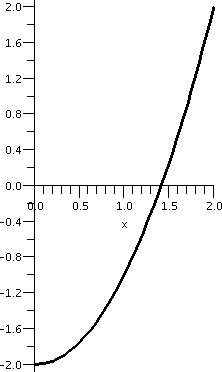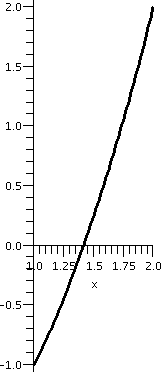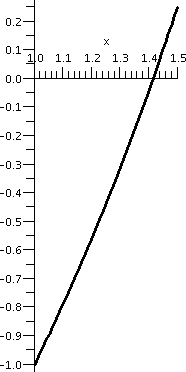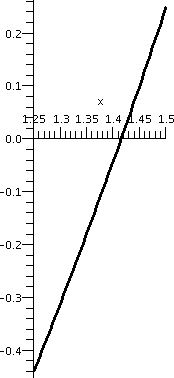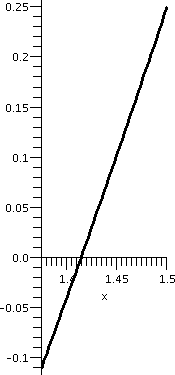The bisection method for root-finding
I discussed this too rapidly and then asked the QotD about it. I regret the hurry. In particular, I mentioned the word "algorithm". Let me give some further information about this word in the form of quotes from The Art of Computer Programming by D. E. Knuth:

The modern meaning for algorithm is quite similar to that of recipe, process, method, technique, procedure, routine, except that the word "algorithm" connotes something just a little different. Besides merely being a finite set of rules which gives a sequence of operations for solving a specific type of problem, an algorithm has five important features:
1. Finiteness An algorithm must always terminate after a finite number of steps.
2. Definiteness Each step of an algorithm must be precisely defined; the actions to be carried out must be rigorously and unambiguously specified for each case.
3. Input An algorithm has zero or more inputs, i.e., quantities which are given to it initially before the algorithm begins. These inputs are taken from specified sets of objects.
4. Output An algorithm has one or more outputs, i.e., quantities which have a specified relation to the inputs.
5. Effectiveness An algorithm is also generally expected to be effective. This means that all of the operations to be performed in the algorithm must be sufficiently basic that they can in principle be done exactly and in a finite length of time \e
Knuth continues on the same page to contrast his definition of algorithm with what could be found in a cookbook:
Let us try to compare the concept of an algorithm with that of a cookbook recipe: A recipe presumably has the qualities of finiteness (although it is said that a watched pot never boils), input (eggs, flour, etc.) and output (TV dinner, etc.) but notoriously lacks definiteness. There are frequently cases in which the definiteness is missing, e.g., "Add a dash of salt." A "dash" is defined as "less than 1/8 teaspoon"; salt is perhaps well enough defined; but where should the salt be added (on top, side, etc.)?
... a computer programmer can learn much by studying a good recipe book

Discussion of the bisection algorithm Specification of the bisection algorithm
The bisection algorithm is one of the simplest and neatest algorithms. It approximates roots very nicely. The entry conditions are a continuous function defined on an interval which includes the interval's endpoints. Also the sign of the function at the endpoints differs: the function's value is positive at one endpoint and negative at the other. Then, according to the Intermediate Value Theorem, there must be at least one root (f(x)=0) inside the interval. Another entry condition is a positive number, here called epsilon, which serves as the error tolerance for the root. Entry conditions
A continuous function f(x) defined on an interval [a,b], with f(a)·f(b)<0;
a positive tolerance epsilon for the error.
Here we check if the interval we're looking at already fulfills the error tolerance condition. In later steps, we will be altering the interval, and shrinking it. Exit condition
If b-a<epsilon, report the interval [a,b] as the answer.
Compute the value of f(x) at the middle of the interval. Computation
Let c=(1/2)(a+b). Compute f(c).
Here's the heart (?) of the algorithm. If f(a) and f(c) have different signs, then the root desired is in [a,c], the left half of the interval. We then change the interval (shrinking it) and see if the length of the interval is small enough. If the signs of f(a) and f(c) are not different, the root we're looking for (whose presence is guaranteed by the Intermediate Value Theorem!) is in the right half of the interval. So we redefine [a,b] as the right half interval and check if the exit condition is satisfied. Decision
If f(a)·f(c)<0, then change b to c, and return to check the Exit condition.
Otherwise, change a to c, and return to check the Exit condition.

### Monday, September 18

Office hours
My formal office hours will be from 10:30 to noon on Wednesday and Thursday. These are times when I will try to definitely be in my office (or, sigh, in the building, at least!). You can certainly talk to me before or after class, or communicate by e-mail, if you'd like to see me at other times. I will most likely be on campus most days.

Workshop work ...
Workshops are important, as I've said previously. And they will be graded (by the recitation instructor and by me) equally for both mathematical content and technical exposition. Please try to do both, and please ask for advice about writeups from either of us.

Textbook homework assignments
The textbook homework assignments to be handed in on a Thursday should be available each week on Monday. I hope this will help you schedule your work.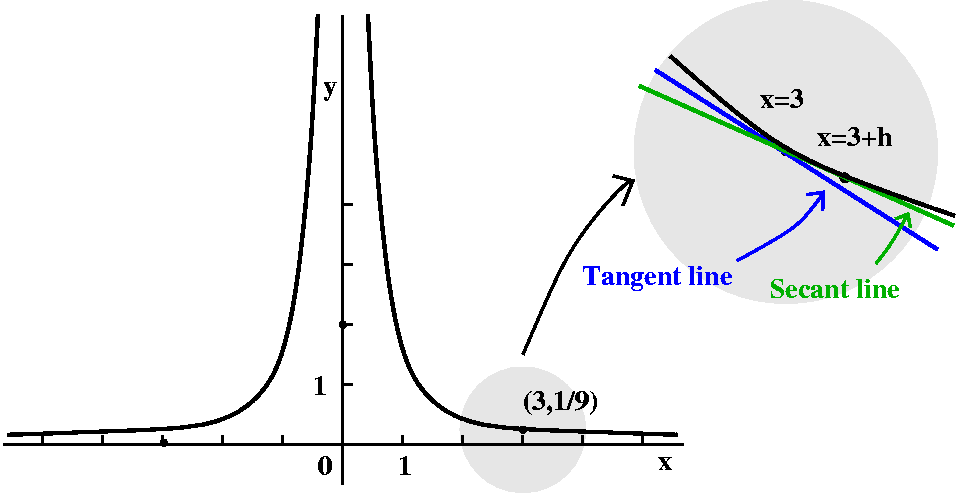Slope of a tangent line
Let's look at the curve y=1/x2 near x=3. The point (3,1/9) is on the curve, since 1/32=1/9. We can try to find the equation of a line tangent to the curve at (3,1/9). Since we know a point this line goes through, we will be able to find an equation for the line if we know a slope. I'll call the slope of the tangent line mtan. The traditional calculus way to find this slope is to approximate it with msec, the slope of a secant line. This will be a line which goes through the point (3,1/9) and (3+h,1/(3+h)2) when h is small. We can get this slope by writing it as the difference in the second coordinates divided by the diffeence in the first coordinates:

```   1      1
----- - ---
(3+h)2 - 9
--------------
(3+h)-3```
I'd like to see what happens when h gets very very small. In the geometric picture the secant line is held down at (3,1/9) and as h gets small, the secant line will sort of revolve into the tangent line, and msec will get close to mtan. Algebraically, if I inspect the quotient I've just written and try to quickly see the behavior as h gets small by replacing h by 0, the result will be 0/0, and we can't assign any value to this quotient. But let's try some simple algebra on the quotient, first replacing the compound fraction by an equal simple fraction:
```   1      1            9-(3+h)2
----- - ---         -----------
(3+h)2 - 9            9(3+h)2            9-(3+h)2
--------------  =  --------------  =  ---------------
(3+h)-3              h                 h9(3+h)2    ```
Now let's "expand" the top and cancel the 9's.
``` 9-(3+h)2        9-(9+6h+h2)        -6h-h2
-----------  =  -------------  =  -----------
h9(3+h)2         h9(3+h)2          h9(3+h)2
```
But we can cancel factors of h from the bottom and from the top (we must cancel an h from both terms on the top).
```  -6h-h2        -6-h
---------  =  ---------
h9(3+h)2      9(3+h)2
```
Now the "asymptotic" nature of the fraction shouldn't be too difficult to see: when h gets close to 0, the top of the fraction gets close to -6 and the bottom gets close to 9·9=81. So I think that mtan should be -6/81, which is -2/27.

Is mtan=-2/27 "reasonable"? Well, if we look at the graph we can see that the y values on the curve are getting smaller as the x values increase (near x=3). So the tangent line should tilt down, which makes the minus sign of the answer more agreeable. The magnitude of the slope, 2/27, is small, and, in fact, if you really look at the curve, the tilt is quite small. Some graphs are shown below. Of course 0 is not in the domain of 1/x2.
y=1/x2; x between -5 and 5 y=1/x2; x between 2 and 4 y=1/x2; x between 2.9 and 3.1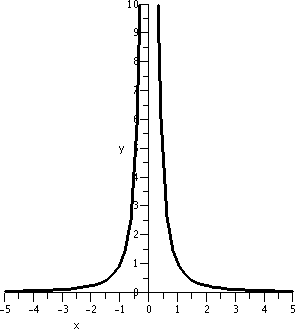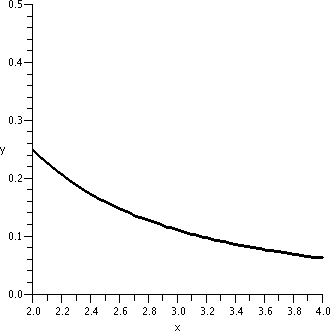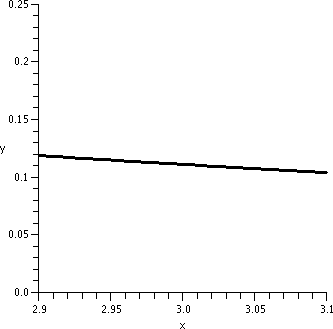The graphs need to be looked at a bit carefully. The vertical interval in the first graph is [0,20]. In the second graph it is [0,.5], and in the third graph it is [0,.25]. This graph is locally linearizable near x=3 in the following sense: if we "zoom in" on it near the point (3,1/9) a sufficient number of times, the graph will look as near to a straight line as we would like. The line that results will got through (3,1/9), and will have slope -2/27.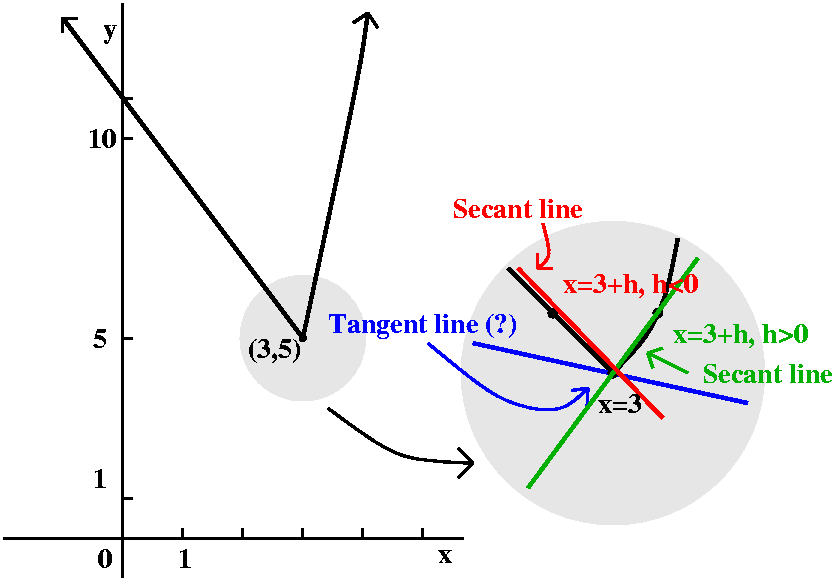Possibly the slope of another tangent line
Suppose now that we define f(x) in a piecewise fashion:

```      11 -2x if x<3
f(x)=
x2-4 if x≥3```
Now the analysis of the slopes of the approximating secant lines is more complicated. A graph of the function (with lots of other "stuff") is shown to the right. The point (3,5) is on the graph, and the point (3,5) also satisfies y=11-2x and y=x2-4. There is no jump or break in the graph at (3,5). Please note, though, that the vertical and horizontal scales differ. Let's analyze msec if h>0:
``` f(3+h)-f(3)      (3+h)2-4-5        9+6h+h2-9
------------  =  ------------  =  -----------  =  6+h
h                h               h```
Therefore as h gets small (with h positive) msec seems to get close to 6. So 6 seems to be our candidate for mtan.

msec if h<0:

``` f(3+h)-f(3)       11-2(3+h)-5        11-6-2h-5
-------------  =  --------------  =  ------------  =  -2
h                 h                 h```
Therefore msec seems to be -2.

There does not seem to be one value of mtan here. So I guess there may not be a unique satisfactory tangent line. Some pictures may help.
y=1/x2; x between -2 and 6 y=1/x2; x between 2 and 4 y=1/x2; x between 2.9 and 3.1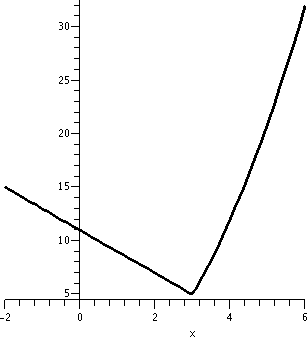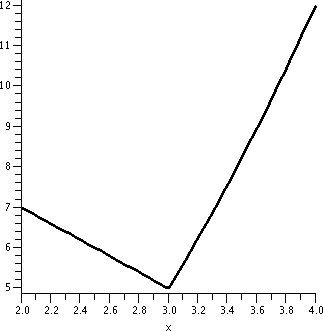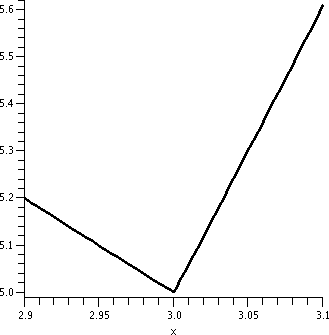Again look closely at these pictures, which all have differing horizontal and vertical scales. In this case, there's no amount of "zooming in" which will make the graph look like a straight line segment. This graph is not locally linerizable. The behavior is qualitatively very different from the previous example.

Limits
The relationship between the approximating msec and mtan needs to be investigated more precisely. There's a name to the process: LIMIT. Limits are fundamental to precise statements about asymptotic relationships of all kinds.Exactness and reality
"Real" functions are not very exact. A chemical engineer might have (I'm simplifying hugely) some process which produces the correct kind of plastic if, say, a certain amount of benzene is used in the mix. (Hey: benzene, with chemical formula X3Y7Z11 (nah), was suggested by a student. So a certain percent of benzene, say 3%, may produce a plastic which has the desired amount of, say, translucence. But in reality, even very precise measurements may not create an input with exactly 3% benzene. Maybe some days we get 3.5, or 4.2. And maybe the desired output measurement is not necessary -- we can deal with some error in the output. Real measurements imply that we need to contemplate error, not as a moral defect (at least in this case), but as part of our mathematical model which we must deal with.

Output tolerance as controlled by input tolerance
So maybe we need to understand the following idea: we want a certain output tolerance: that is what we can "live with" in the material created. Is there an input tolerance describing inputs in an interval around the ideal input which makes the corresponding outputs close enough to the desired output? This is too darn abstract. Let me consider a numerical example, with a very simple function.

x2 near x=3
 If the input to f(x)=x2 is 3, the output will be 9. Suppose we are willing to live with a +/- error of 1 in the output. That is, we can tolerate |f(x)-9|<1. Is there some simple specification of an interval of inputs near 3 which will guarantee this? In this case, almost everyone thinks that the simplest kind of input specification would be an interval of x's centered at 3 (your feelings may differ here, but this is what is usually done). So I ask if there is some convenient number ("CN") so that if |x-3|

Change the output tolerance to .2
What if we changed the desired output tolerance to .2? That is, we want to get |x2-9|<.2 by controlling the size of |x-3|.
 Here's the picture of f(x)=x2 with the two horizontal red lines indicating the output tolerance needed.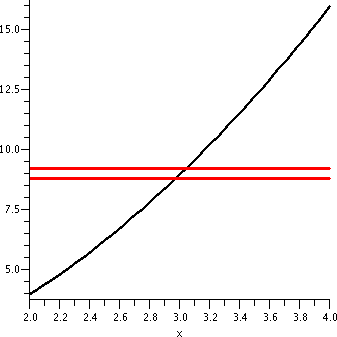Now here is the "old" input tolerance lines which we just used. Please note that this input tolerance won't work: there is part of the graph of x2 between 2.9 and 3.1 which is not between y=9-.2 and y=9+.2.1.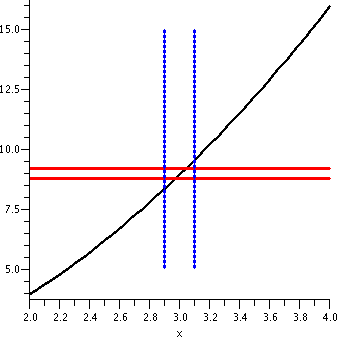Here is a display showing a satisfactory input tolerance. I used .1 as the input tolerance (yes, I experimented a bit). I repeat that in reality people rarely care about the "best possible" input tolerances. They usually just want to get some number that works. To me this graph doesn't show very much. So let's try a different scale.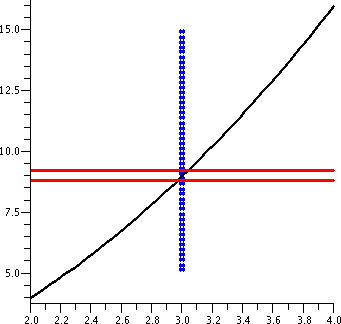I hope that you can see here a graphical "verification" of the algebraic implication:      If |x-3|<.01 then |x2-9|<.2 An input tolerance of .01 satisfies the output tolerance of .2.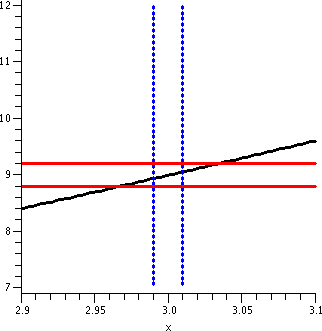x2 near x=10
Now let me change the game a bit. Suppose I want to consider the same function, f(x)=x2, but now investigate what happens near 10. Since f(10)=100, the "ideal" input is 10 to get the "ideal" output of 100.
 Suppose I want the outputs to be within 1 of the ideal output. That is, I want |x2-9|<1. The horizontal lines shown indicate this restriction. Warning! There is a huge horizontal/vertical distortion in this graph. The horizontal scale ranges from 9.5 to 10.5, a length of 1. The vertical scale goes from about 90 to about 110, a length of 20. The "true" picture is a long and thin vertical rectangle, with a 1-to-20 aspect ratio.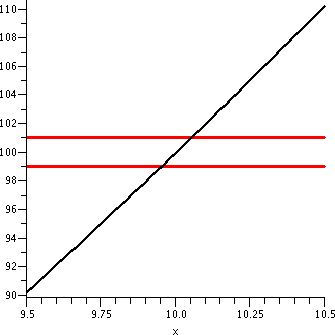The vertical lines shown are x=9.9 and x=10.1, which would be the geometric side of the input restriction |x-10|<.1, the input tolerance we used in "If |x-3|<.1 then |x2-9|<1" The same input tolerance won't work about different "ideal inputs". If you really believe in the graph, this isn't too surprising since the graph is much more tilted around x=10 than it was around x=3.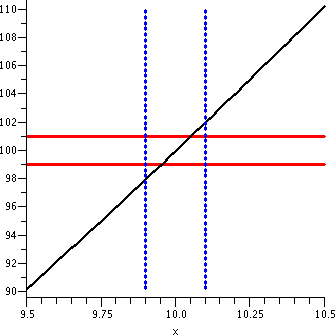The vertical segments shown are parts of the lines x=9.97 and x=10.03. I hope this is pictorial evidence that the following implication is correct:      If |x-10|<.03 then |x2-100|<1 An input tolerance of .03 satisfies the output tolerance of 1 near the ideal input/output pair (10,100).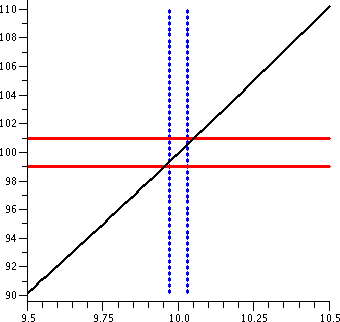Complicated? Yes, it is.
These pictures should convince you that the limit business when addressed "officially" is indeed complicated. In your courses and careers, there may rarely be times when you'll need to cope with these implications. But I believe you should have some idea of what's going on.
Graphs don't really work too well for complicated functions or irritating input/output pairs. People have therefore developed extremely intricate strategies relating the inequalities needed. Serious investigation of this algebra is not the aim of the course but the equipment is there when/if you need it.

The official definition
The statement limx-->af(x)=L means:

Given any (positive) output tolerance, there is a (positive) input tolerance so that
If 0<|x-a|<the input tolerance, then |f(x)-L|<the output tolerance.
The traditional algebraic abbreviations for "output tolerance" and "input tolerance" are the Greek letters epsilon and delta. So the definition above is frequently called the epsilon-delta definition or criterion.
I also slipped in a slight change: on the input side, what's needed is "{ 0<|x-a|<the input tolerance". The 0<|x-a| requirement was not mentioned before. That's put in primarily because the most immediate use we will make of limits will be to compute slopes of tangent lines, what are called "instantaneous rates of change". In that use you should see already that we sometimes will not be able to just "plug in" the ideal input (otherwise we'll get a 0/0 situation). So plugging in the ideal input is NOT part of the definition!

### Wednesday, September 13

Diary entry in progress!

The piecewise linear function

The inverse(s)

Example

Some trig functions and their inverses

Some exponential functions and their inverses

Dwarf apple trees

Growth rate

Ultimate height

Chapter 2 and its fictions

Slope of a tangent line

QotD

### Monday, September 11

More background
I discussed some of the purposes for some of the things I will do in this course.

The diary
I mentioned the diary and remarked that it served several purposes. First, even very enthusiastic students may sometimes miss a class. The diary can help them find out what was covered. Another reason for the diary's existence is so that I can fix errors which I will certainly make, so while the diary will be a representation of what went on in class it won't be a totally accurate one: improvements may occur! Also, even if I don't make a mistake in the lecture, sometimes I may not explain things optimally, so the diary may give students another chance to understand the presentation. I really don't think that a diary entirely takes over the teaching/learning aspect of the lectures. There is still a good amount to be said about the interaction between the instructor and the student, provided that students are willing to ask questions and (sigh!) the instructor is really willing to listen and answer. I will try to do the latter (also with errors, of course, since I plead the defects of humanity!).

QotD

Study groups
I asked students in the class to tell me their e-mail addresses and likely majors, and, with appropriate permission, this information is now displayed on a web page. Now at the beginning of this course, there may be little that is new to students. I caution you, though, that even if you have seen much of this material before, the course will move swiftly. It is a good idea to form study groups meeting at appropriate times and durations to go over homework, etc. I hope that the student list will help people get in touch with each other.

Calculus originated as a way of describing certain methods used in understanding and solving problems originating in geometry and physics. The language of the subject shows this ancestry. Calculus has been very successful. Calculus also "happens" to be suitable to describe and study many problems in biology and economics. Any problems which involve rates of change (how soluble is substance A in substance B as the concentration of substance C varies?) or accumulation of changes (what is the present value under certain interest rate assumptions of a stream of mortgage payments to be made monthly over the next 20 years?) is a problem which likely can be described using calculus. Frequently this description will suggest certain ways of solving the problems using the methods of calculus. That's why the subject is required in so many majors.

Functions
The word function is used in a technical sense in calculus, and is one of the most important vocabulary words. It is the logical setting for how things are transformed to other things. In the case of Math 151, the "things" are numbers. So functions will change numbers to numbers. I decided to begin with what I hoped was a known example described verbally.Hooke's law
Think of a spring with a rest or "equilibrium" position. Then Hooke's Law states that the deviation from the rest position is in direct proportion to the impressed force. There are many assumptions involved in Hooke's Law, but to a considerable extent it is a widely valid shorthand for a great variety of real phenomena. If I further add something like: the spring gets stretched 10 inches by a weight of 300 pounds, then I can formulate an equation relating the impressed force and the deviation from the equilibrium position:
D(x)=(1/30)x
Here D(x) is the deviation in inches from the equilibrium position (with positive meaning the spring is forced to be longer) and x is the impressed force in poinds (I'm thinking maybe of a thick mechanical spring or something like that). x is called the independent variable and D(x) is called the dependent variable. Notice that sigh matters. If x is negative, then we could be compressing the spring, and the end of the spring is higher than the equilibrium position in our model.
Notice that as in all mathematical models, you need to be alert a bit about how "correct" this is. Uhhh ... if we stretch a rubber band with a force of six trillion tons, I don't necessarily think it will end up having a length of one lightyear.

FunctionsOne metaphor I will use (and probably overuse!) in this course is the idea of a function as a machine with an input and a unique output associated to each input. The collection of all valid inputs to the machine, those inputs which don't cause the machine to break, is called the domain. The collection of all of the outputs for these valid inputs is called the range.

Functions via formulas
Functions can be described simply with formulas. Actually, most of the functions we will study in this course will have such descriptions. Warning: students interested in obviously experimental disciplines (engineering, physics, chem, etc.) will mostly encounter functions which are described by data sets, and must work with them using the techniques of this course which will mostly be presented using functions defined by formulas. This can be ... irritating.
The domain of a function defined by a formula is usually assumed to be what's called the natural domain: this is the collection of all inputs of real numbers which make sense in the formula. But there will be exceptions (see below!).

• Polynomials
Polynomials are sums of constant multiplies of monomials. So on example of such a function is f(x)=53x40-9.7x2+88. Polynomial formulas only involve multiplication and addition, and therefore the natural domain of a polynomial is all real numbers.
Exception!
Already, even in such a simple setting, we can have exceptions. Let's consider the following paragraph, which is really the beginning to a typical "toy" calculus problem:Equal-sized squares are cut from the corners of an 8 inch by 11 inch rectangular piece of paper. The flaps in the resulting piece of paper are folder up. Write a formula for the resulting volume which the paper object encloses, using the edge length of the squares as the independent variable. What is a useful domain for this formula?
I hope that the accompanying illustrations are helpful. I'll call the edge length x. Then the "solid" brick will have volume, V(x), which will be the product of the area of the base multiplied by the height. The height is x, and the base has sides with lengths 8-2x and 11-2x. So V(x)=x(11-2x)(8-2x). This formula is "just" a polynomial of degree 3. But please notice that x=-50 or x=200 makes no sense in this problem. The physically reasonable domain, the x's that can actually be used, are just those x's in the interval [0,4]. So already here is an example of a function given by a formula where the applicable domain for the mathematical model is much smaller than the natural domain. You may think that this is unreasonable, and I'm being much too picky. But the restriction is essential.
I was asked by one alert student to describe the range of V(x). I refused and said I'd need to wait a week or two until we officially had the correct technical equipment. Until then, I recommended that many sheets of paper have squares cut from them, many little containers be created, and then measure the volumes (fill with water or maybe popcorn?).
• Rational functions
These are quotients of polynomials, for example f(x)=(x4+6)/(x2-5). Since we are now "allowed" to divide, we'd better avoid dividing by 0. Here we need to find out where x2-5=0 which is x=+/-sqrt(5). So the domain is all other x's: the intervals (-infinity,-sqrt(5)), (-sqrt(5),sqrt(5)), and (sqrt(5),infinity). Things can get complicated, especially because if you give a "random" polynomial, finding the roots exactly may not be possible!
• Algebraic functions
Here we allow roots to occur, so we can get things like f(x)=sqrt(x2-5). Since even powers can't be negative, we'd better have inputs to square roots (and other even roots) be non-negative. So here we need x2-5≥0, which means that the domain needs to be (-infinity,-sqrt(5)] and [sqrt(5),infinity).
• Exponentials and logs
We'll deal more with these in the future, but exponentials are always positive, so the domains of logs have restrictions to only positive numbers. These functions are needed to model many sorts of growth problems.
• Trig and inverse trig functions
So we need to consider sine and cosine and tangent (domain restricted to not allow division by 0) in order to model periodic phenomena. The inverse trig functions have various kinds of domain restrictions.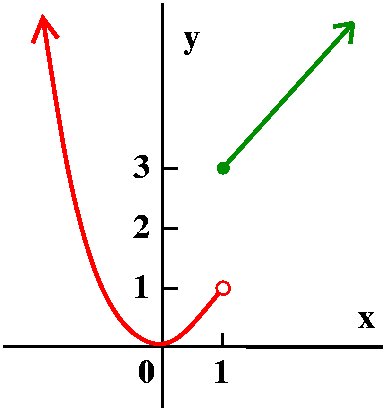Piecewise defined functions
Here is a silly example:

```      x2 if x<1
f(x)=
x+2 if x≥1```
Such examples will be useful to consider when we look at limits. Here f(-2)=(-2)2=4 and f(5)=5+2=7.

Part of the graph of this function is shown to the right. Please note the "empty circle" on the parabolic arc, because of the restriction x<1 (x is not allowed to be equal to 1). There's a "filled circle" on the other piece, a half line.

A more elaborate "real" example
Now here is a somewhat more complicated example:

```f(x)=
.1x if 0≤x≤15,100
1,510+.15(x-15100) if 15,100<x≤61,300
8,440+.25(x-61300) if 61300 <x≤123,700
24,040+.28(x-123700) if 123,700<x≤188,450
42,170+.33(x-188450) if 188450<x≤336,550
91,043+.35(x-336,550) if 336,550<x
```
This is the U.S. individual tax rate schedule (form 1040) for 2005. The function has many weird numbers in it. This is an example of a piecewise linear function because each of the pieces is defined by a polynomial of degree 1. The slopes of the pieces increase as the x's increase because this is a progressive income tax. But the other strange numbers are caused by a desire to avoid jumps and breaks in the graph of the tax function, so that strange situations don't arise (examples: yes, you earn \$1 more and therefore will pay \$100 less in taxes, etc.).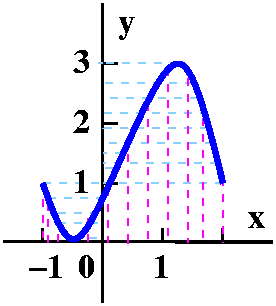Graph of a function

The graph of a function is the collection of all points in the plane with coordinates (x,f(x)) if x is in the domain of the function. To the right is the graph of a function. A collection of points in the plane is the graph of a function if every vertical line which touches the graph intersects it exactly once. This is called the Vertical Line Test, and corresponds to the fact that we want exactly one output determined by every "legal" input. If we have the graph, the domain is all x's on the x-axis for which the vertical line through that x hits the graph. Similarly the range is all y's on the y-axis for which the horizontal line through that y hits the graph. Sometimes if you only have the graph and no other information, precise descriptions of the domain and range can be difficult.

 An inverse function Consider the function defined by f(x)=2x3+7. Part of the graph of this function is shown to the right. It was produced by my silicon friend, Maple. (Available on eden, just type maple). The graph is a bit deceptive. I asked that the function be shown for x's in the interval from -3 to -3. The program automatically "autoscales" and squeezes so that the image is in a square. This can be confusing at times if you don't realize it. In this case, the vertical range seems to be between -45 and 60.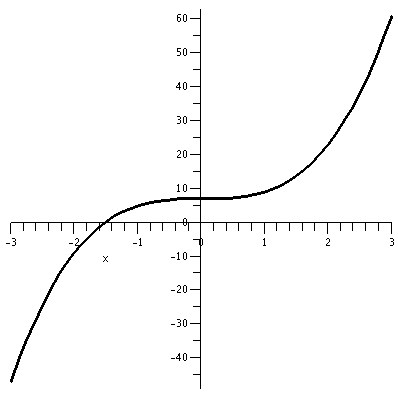The part of the graph I want to emphasize here is the qualitative aspect: certainly every vertical line hits the graph once (the Vertical Line Test) but so does every horizontal line. That is, every output corresponds to a unique input. For this function, this uniqueness claim can be verified algebraicaly quite easily: if w is an output, we can write a formula for the unique x it came from. w=2x3+7 --> w-7=2x3 --> (w-7)/2=x3 -->[(w-7)/2]1/3=x A function which has unique outputs for each input is called 1-to-1. Such a function has an inverse. The function g(w)=[(w-7)/2]1/3 is inverse to f. It undoes f, so that f(g(w))=w and g(f(x))=x. The graph of g is shown to the right. It is gotten algebraically by interchanging the two coordinates of each point. Geometrically the graph of the inverse function is gotten by picking up the original graph of f and "flipping it over" the main diagonal, y=x.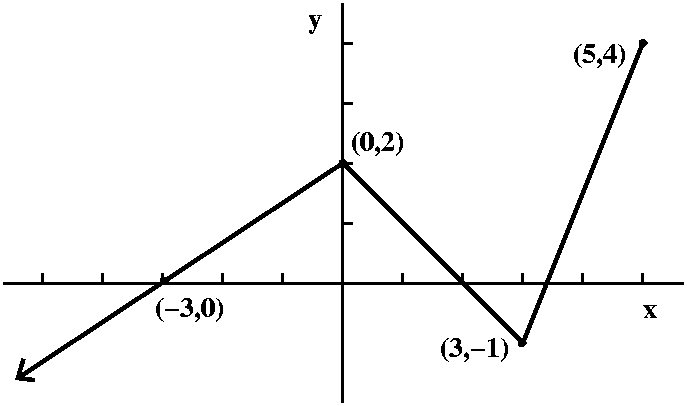A piecewise linear function to play with
I defined a function by sketching its graph, as shown to the right. I declared that the function, f(x), was piecewise linear. One part was a half infinite line (a ray) passing through (-3,0) and ending at (0,2). Then there was a line segment from (0,2) to (3,-1), followed by a line segment from (3,-1) to (5,4). The domain of this function was (-infinity,5] and its range was (-infinity,4]. My real intention was to use this function to study the problems involved in specifying inverses, but ...

QotD
Suppose H(x)=f(x2) and K(x)=f(x)2. I asked students to tell me the domain and range of H and K. Below, by the way, are graphs of f and H and K, as Maple "sees" them. Notice again that vertical and horizontal scales differ.

Graph of part of fGraph of all of HGraph of part of K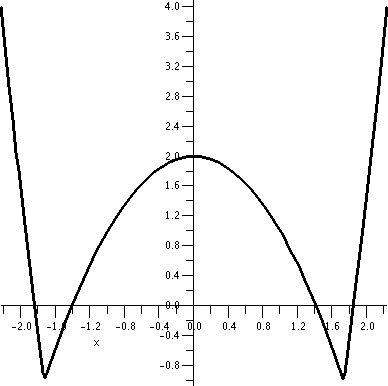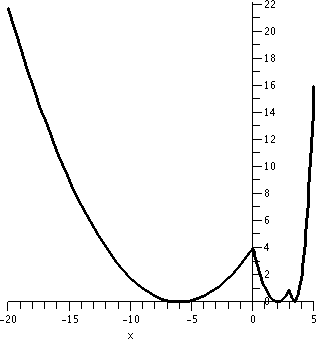Let's see: H(x)=f(x2). The inputs to f are (-infinity,5]. But squaring makes things non-negative, so the only numbers I can push into x so x2 feeds into f are numbers which won't square to bigger than 5. Therefore I should put in only numbers between -sqrt(5) and +sqrt(5). So the domain will be [-sqrt(5),+sqrt(5)]. The range will be the outputs of f when the inputs of f are in the interval [0,5], and those outputs are [-1,4].
K(x)=f(x)2. Now K has the same domain as f: (-infinity,5] because the domain of squaring is every possible number -- there are no restrictions to worry about. The outputs, however, are squared. The collection of outputs of f(x) are (-infinity,16]. When these are squared we will get every non-negative number.
Here is more detail. For x>0, the outputs of f(x)2 go from 0 to 16. On the left, though, the outputs of f itself go down to -infinity, so squaring means the results are all non-negative numbers. The range of K is [0,infinity).

### Wednesday, September 6

We wasted some time giving an "exam" which will be used to help the math placement process. I believe this is a good thing although I disliked using class time.

Numbers

• Positive integers
We can start with 0 (the "additive identity": anything plus 0 is that anything) and 1 (the "mulitplicative identity": anything times 1 is that anything). 1's added to 1's create the positive integers.
• Integers
If subtraction is considered, then we need "additive inverses". The additive inverses of the positive integers are negative integers. These together with 0 are all integers.
• Rational numbers
Now multiplicative inverses force the consideration of reciprocals of integers (except for 0, because that would lead to real algebraic difficulties). Further multiplication makes the collection of rational numbers.
• All the others (these are most of them!)
Rational numbers have finite or repeating decimal expansions. In this course we will use various sequential approximation processes, and we will need to consider numbers with all sorts of decimal expansions, not just repeating expansions. These weird and "chaotic" numbers are not rational: they are irrational. In various ways which can be made quite precise, there are many more irrational numbers than there are rational numbers: a typical number is irrational. Any physical experiment or process when measured gives only an approximation to some sort of "true" answer, and this sequence of approximations, like the decimal expansion, is likely to be an approximation of an irrational number.

Geometry
The real numbers are usually thought of as corresponding to a specific geometric object, the real line. I usually think of this line as horizontal with 0 sitting "in the middle". 1 is to the right of 0. And this geometric picture brings up the idea of order. In addition to the algebraic structure, there is order: a<b. To me this means (in my picture of the line) that a is to the left of b. Negative numbers are to the left of 0 and positive numbers, including all the positive integers, are to the right of 0. Here are some interesting aspects to note.

The decimal expansion of a real number provides an "address" to locate the number geometrically. For example, 23.4680472... means we first move right from 0 by 23 steps, each 1 long. Then look between 23 and 24, and divide that length in tenths. The number we're looking for resides (?) in the 4th subinterval. Now divide that subinterval into tenths. The number is in the 6th subinterval of that collection. Etc. The "Etc." of course conceals an approximation process which will locate the real number with the specified decimal expansion.

Some real numbers have two valid decimal expansions. This doesn't bother me, since, say, a house on a corner of a grid system of streets could also possibly have two valid addresses (if it is on the corner of Cedar Avenue and Third Street, the house could have the address 12 Cedar Avenue and also 42 Third Street). So, for example, the real number with decimal address 23.45999... (with the 9's repeating) also have the decimal address 23.46, a terminating expansion (there are a string of 0's which are usually not written.

Distance including a discussion of | |
The distance between two points is a non-negative real number whose size expresses how far apart the numbers are. This will be important when we study approximation schemes. We'd like to know that the approximation gets "close" to the correct answer, and the closeness will be measure by the distance. Algebraically, if the points correspond to the real numbers a and b, the distance between them is |a-b| and this is the same as |b-a|, so that distance has some symmtery. But I just used absolute value, and here is the piecewise definition of absolute value:
|x|=x if x≥0 and |x|=-x if x<0
Therefore absolute value is always a non-negative number. The absolute value of a number is 0 only when the number itself is 0. And absolute value of a product is the product of the absolute values (this actually is not totally obvious, and needs a bit of thought, I believe).

Intervals
Suppose we want to discover what numbers x are closer to 9 than a distance of 4. Algebraically this requirement translates to |x-9|<4. We can sort of "unroll" the inequality. The absolute value will be less than 4 if the number itself is both less than 4 and greater than -4. The two inequalities can be compactly written as follows:
-4<x-9<4 which implies
5<x<13
This is an interval and an interval which does not contain either endpoint is called an open interval. The notation for this interval is (5,13). Intervals which contain both endpoints are called closed. An examples of such an interval is [-4,6], which means the numbers x satisfying -4≤x≤6. There are also half-open intervals, unbounded intervals (with notation using + or - infinity), etc. Please see the textbook.

Warning!
If you wanted to "solve" (better: understand!) the inequality |x-9|>4 you can't just "unroll" it to -4<x-9>4. This inequality has no solutions. There is no number which is simultaneously less than -4 and greater than 4. You can't write this so compactly and using such implications represents an invalid (wrong!) method of solution.

A valid method of solution would involve separately solving the inequalities:
-4<x-9 orx-9>4 which gives 5<x or x>13. This is actually, therefore, two intervals:
(-infinity,5) and (13,infinity). So the inequality |x-9|>4 has a solution set which is two intervals.

The plane
The conventional way to describe the plane algebraically is to drop down two lines perpendicular to each other: coordinate axes. A point in the plane will then be described by an ordered pair of real numbers. The first coordinate will usually be called the x-coordinate and the second, the y-coordinate. This pair describes ordered distances from the horizontal (the x-axis) and vertical (the y-axis) lines. Please see the text for more about this.

The embarrassment of all this, especially with "new" students, is that (3,8) could describe both a point in R2, the plane, and could also describe an open interval (with [missing!] endpoints 3 and 8). The context is supposed to help, but still the notational confusion is possible, and this is lousy.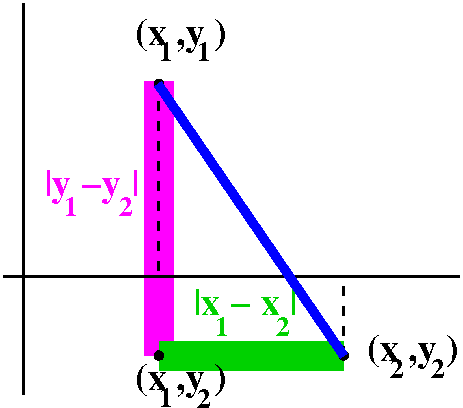Distance in the plane, R2
Well, I went through the usual diagram to try to motivate the algebraic definition of distance in R2. Look, please, at the diagram to the right. In the plane, points correspond to ordered pairs of numbers. So a point p might correspond to an ordered pair, (x1,y1), and q might correspond to (x2,y2). Then the point (x1,y2) is the vertex of a right triangle whose hypotenuse is a line segment connecting p and q. One leg of the right triangle is on a line where all the first coordinates are x1, and the length of that leg is given by the one dimensional formula, |y1-y2|. The other leg is on the line where all the second coordinates are y2, and the length of that leg is |x1-x2|. Then by Pythagoras, the hypotenuse has length sqrt(|x1-x2|2+|y1-y2|2). And usually the absolute values are discarded since we are squaring the quantities. Therefore we officially define:
dist(p,q)=sqrt((x1-x2)2+(y1-y2)2) if p has coordinates (x1,y1) and q has coordinates (x2,y2).Example
The distance between between (3,-2) and (6,4) is sqrt([3-6]2+[4-(-2)]2)=sqrt(32+22)=sqrt(13).

A circle and its algebraic description
Suppose we wanted to describe algebraically the collection of all points which have distance sqrt(13) from (6,4). This is, of course, a circle of radius sqrt(13) and center (6,4). Well, if (x,y) is such a point, then sqrt([x-6]2+[y-4]2)=sqrt(13). This does describe the circle. Of course, if you must, all sorts of algebraic things could be done to the equation. But I am the laziest person in the room, and therefore ...

A (non-vertical) line and its algebraic description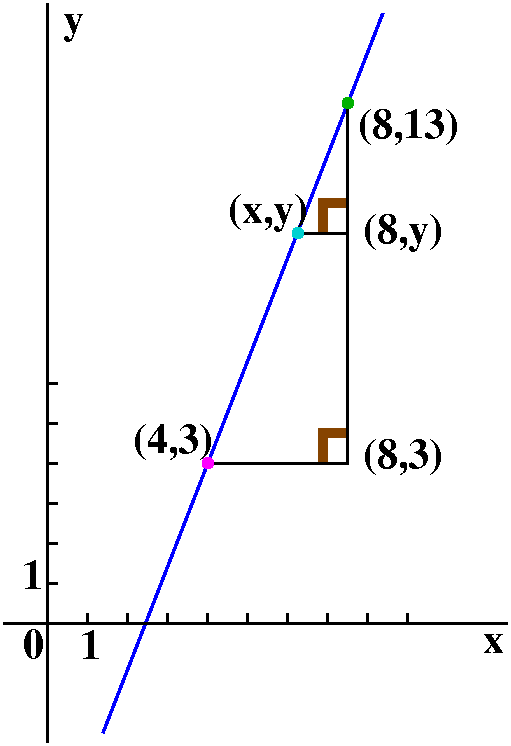Suppose we wanted an algebraic description of points with coordinates (x,y) which lie on the straight line which goes through (4,3) and (8,13). If (x,y) is such a point, then look at the picture: two right triangles indicated are similar, so the corresponding sides have the same ratios:

```13-y   13-3
---- = ----
8-x    8-4```
and the point (x,y) is on the line exactly when y-13=([13-3]/[8-4])(x-8). The quantity ([13-3]/[8-4]) is called the slope, and multiplies changes in x to give changes in y. It is frequently designated with the letter m.

QotD
I gave two points and asked for an equation for the line containing the two points. Most people were able to do this.

Maple
Much intricate algebraic computation can now be done by computer programs. A course like Math 151 can therefore comcentrate more on understanding rather than elaborate computational skills. On the other hand, knowing what results should look like (if you calculator declares that 56.007 is the sum of 1,345 and -14,891, do you believe it?) is important, so a secure command of various algebraic algorithms is still important. At Rutgers, the program Maple is widely available since there is a university license for it. Students may also get copies under certain circumstances. Basic information about the program is here and here are some local help pages.